## Thursday, January 03, 2019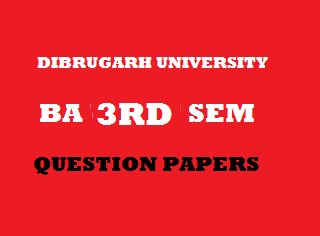2013
(November)
MATHEMATICS
(General)
Course: 301
[Group – A: Coordinate Geometry and
Group – B: Analysis – I (Real Analysis)]
Full Marks: 80
Pass Marks: 32
Time: 3 hours
The figures in the margin indicate full marks for the questions
GROUP – A
(Coordinate Geometry)
SECTION – I
(2- Dimension)

1. (a) Transform the equation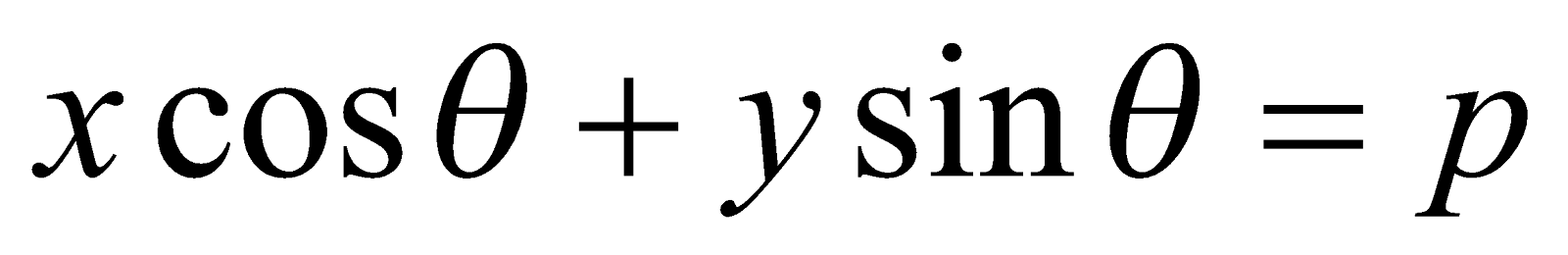by changing to parallel axes of coordinates through the point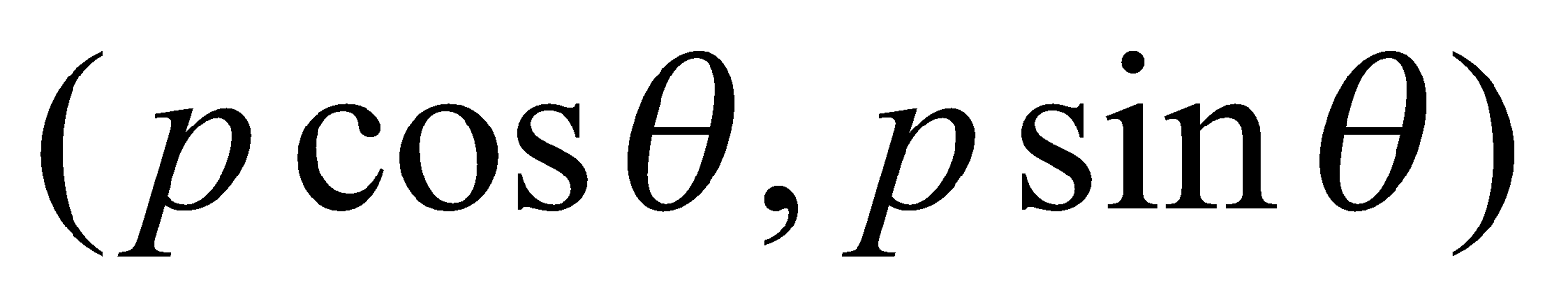. 2
(b) What will be the transformation of the equation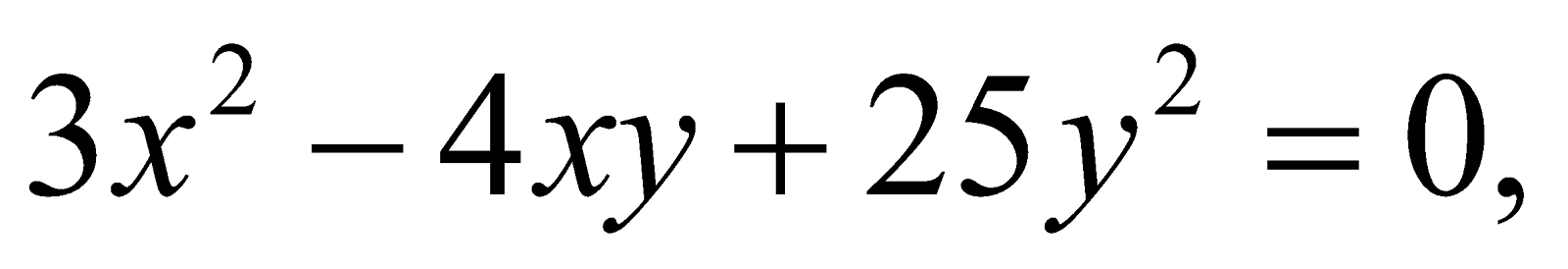when the two axes are turned through an angle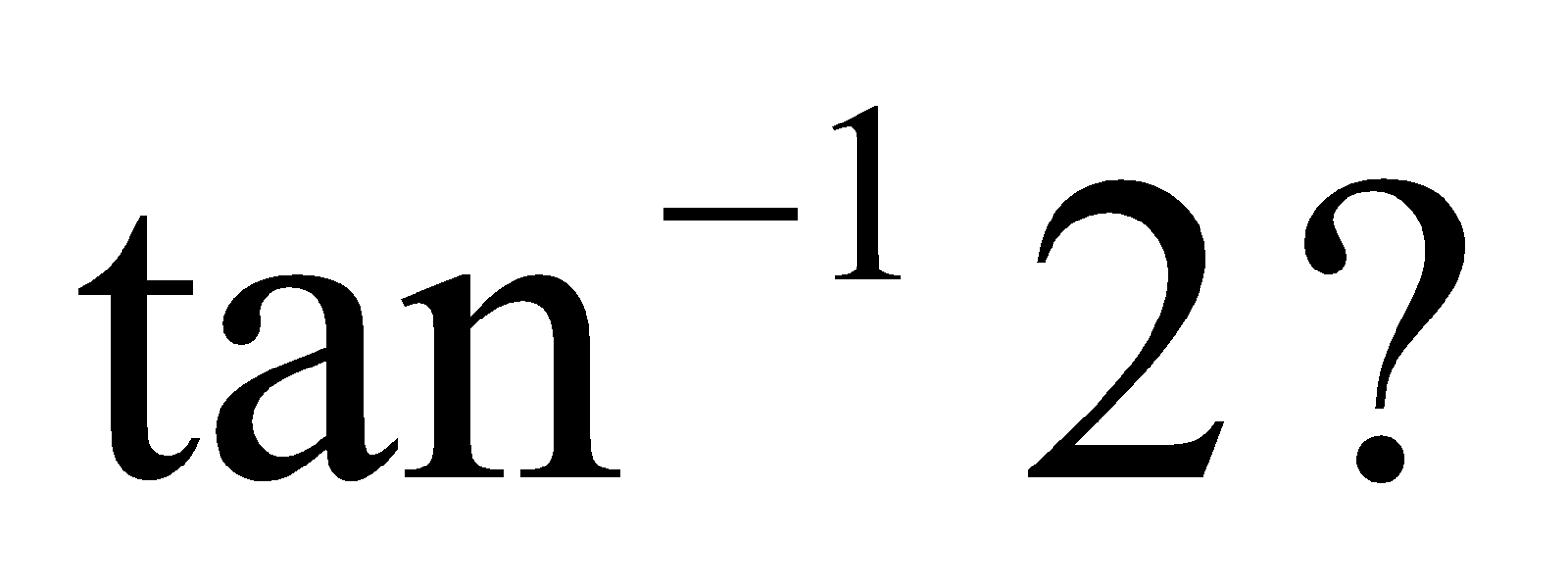Or
To which point the origin will be shifted, so that the first degree terms in the equation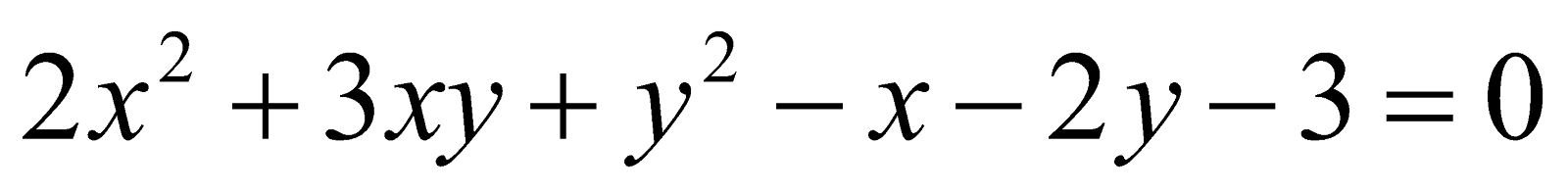may be missed, when referred to the parallel displacement of the axes?
2. (a) Find the angle between the two lines represented by the equation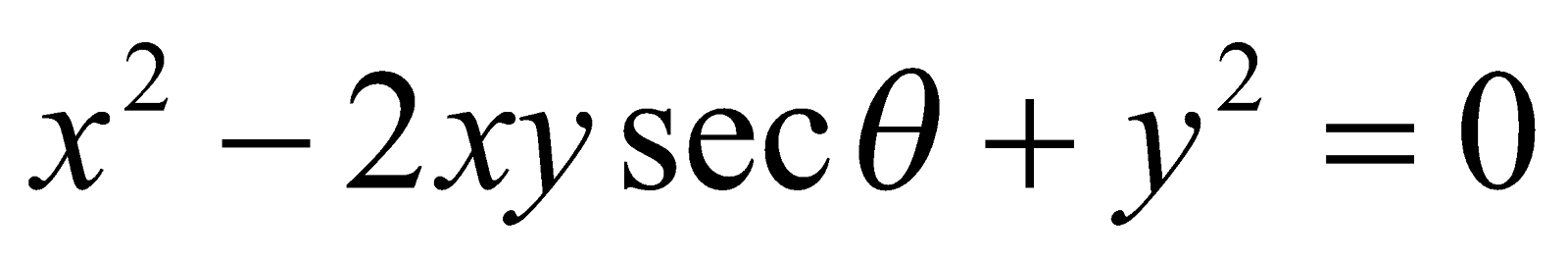(b) Determine the distance between the two parallel lines represented by the equation 3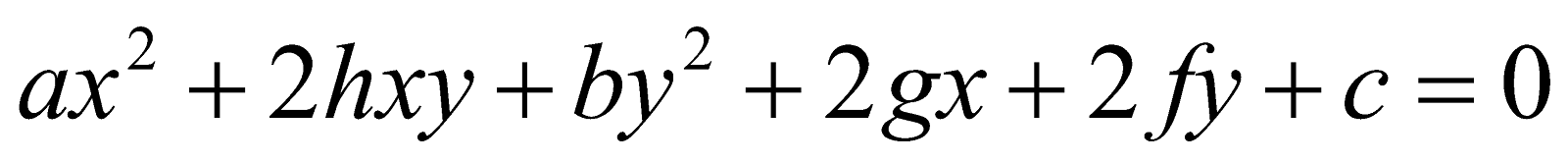Or
For what value of k the equation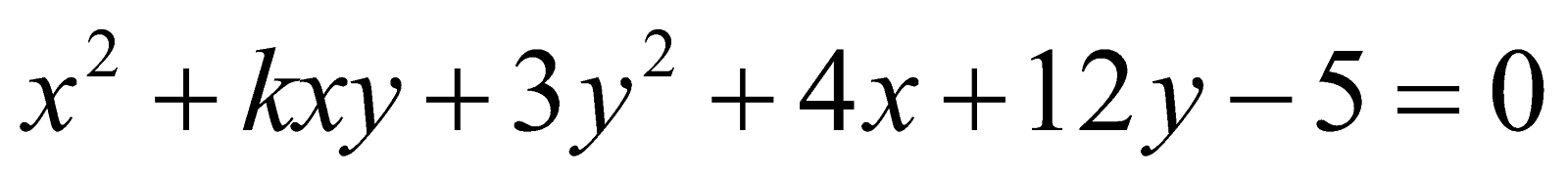(c) Show that the two straight lines represented by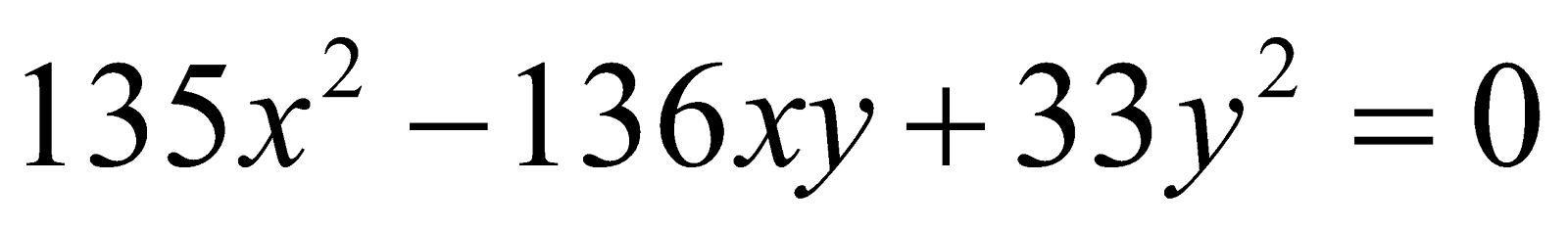are equally inclined to the straight line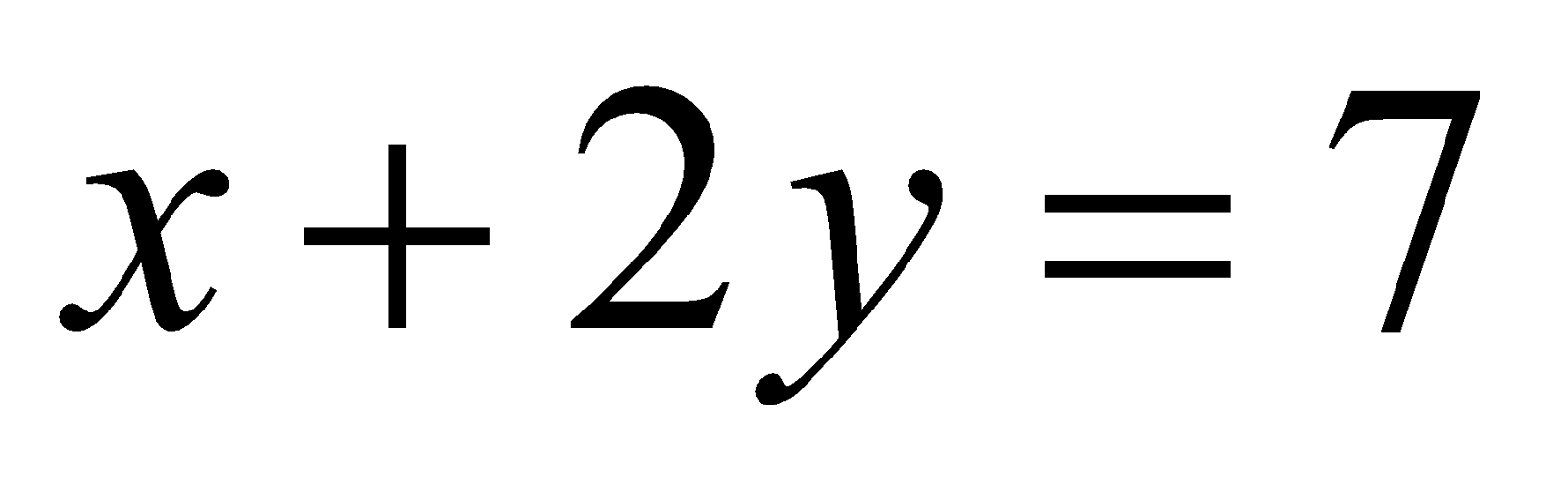. 3
(d) Prove that the product of the lengths of perpendiculars drawn from the point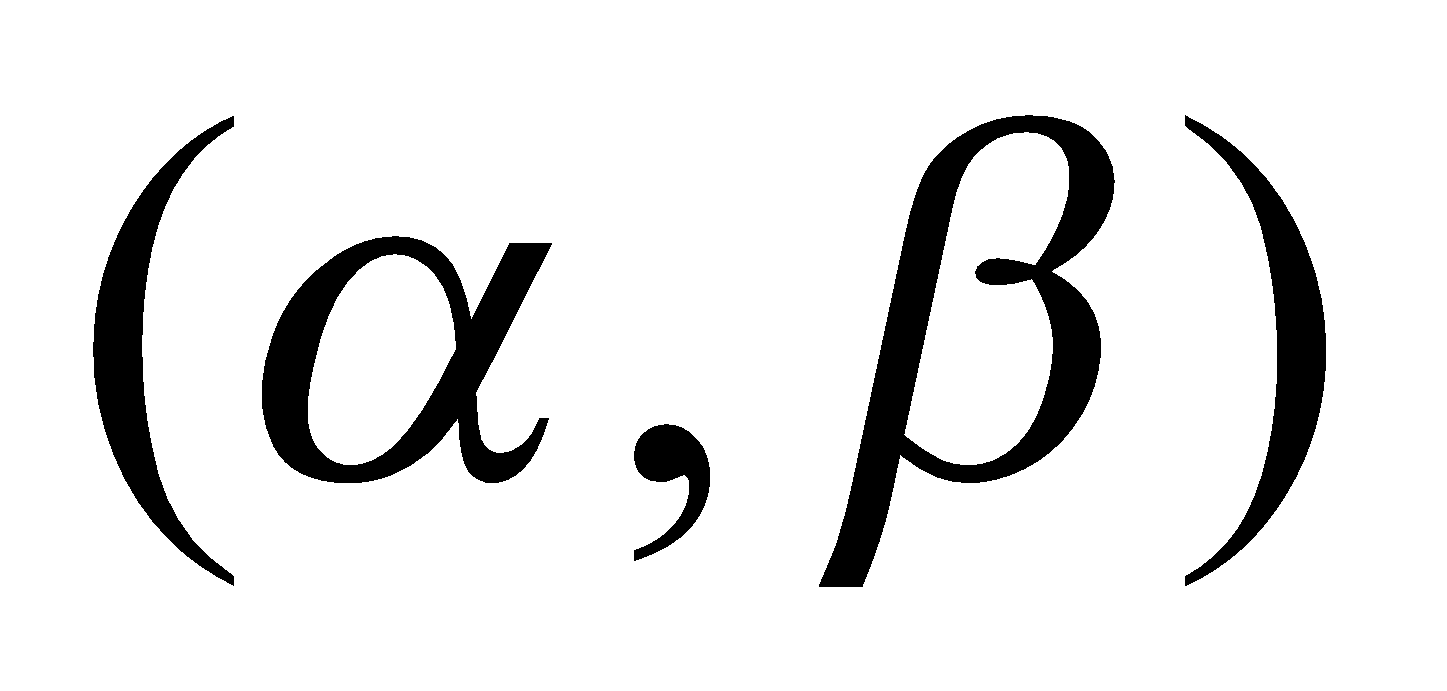to the line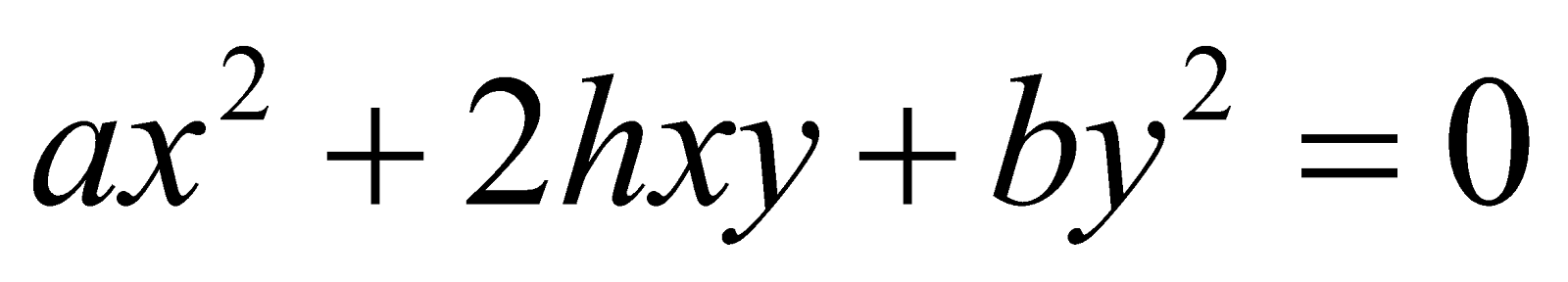is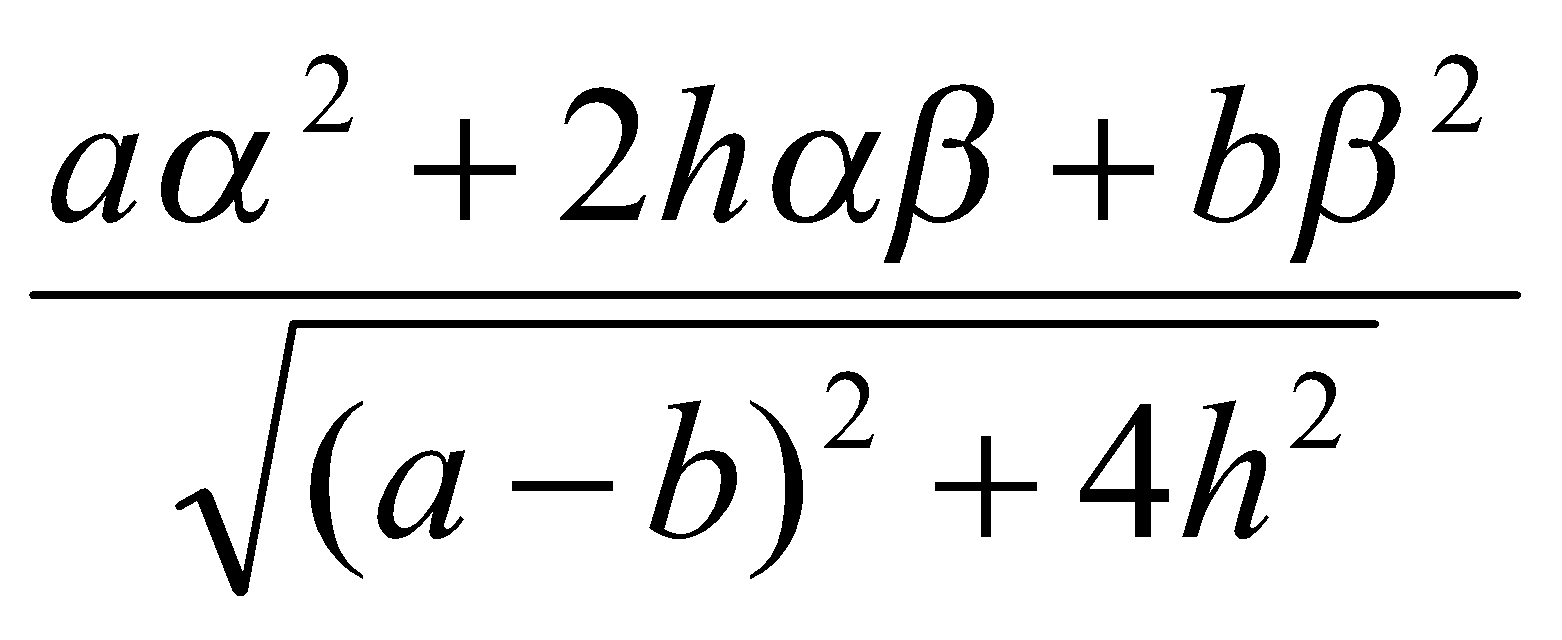Or
Find the area of the triangle formed by the lines represented byand axis of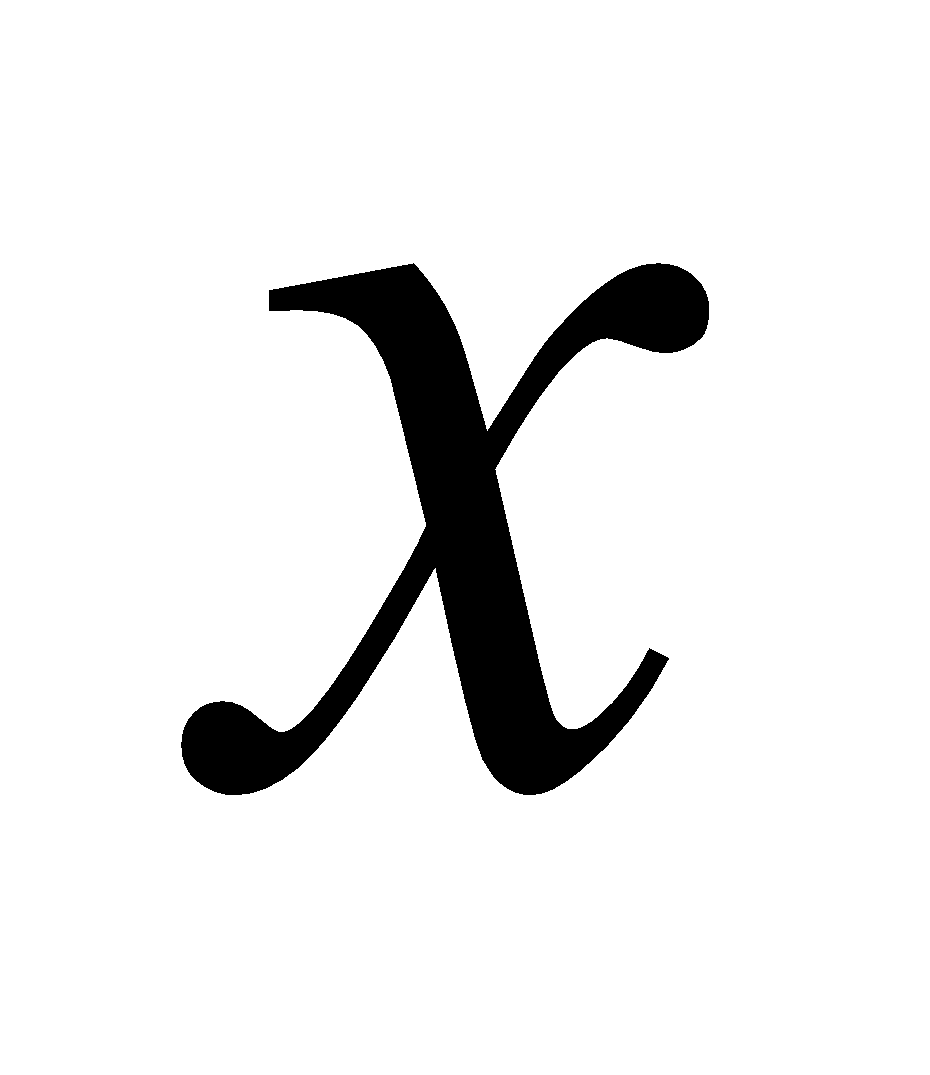.
3. (a) Reduce the equation 2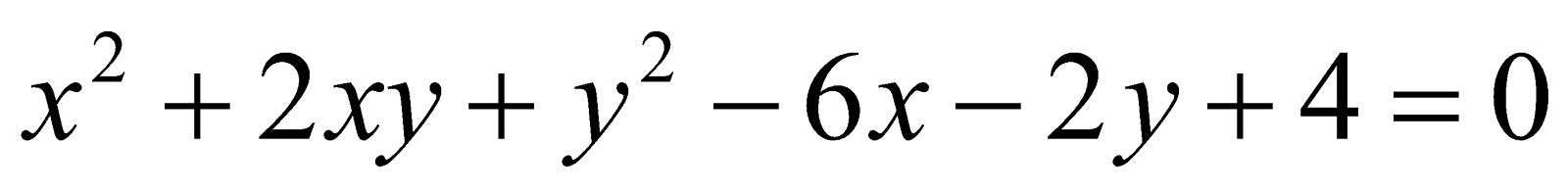into standard form.
(b) Find the equation of the tangent drawn at the point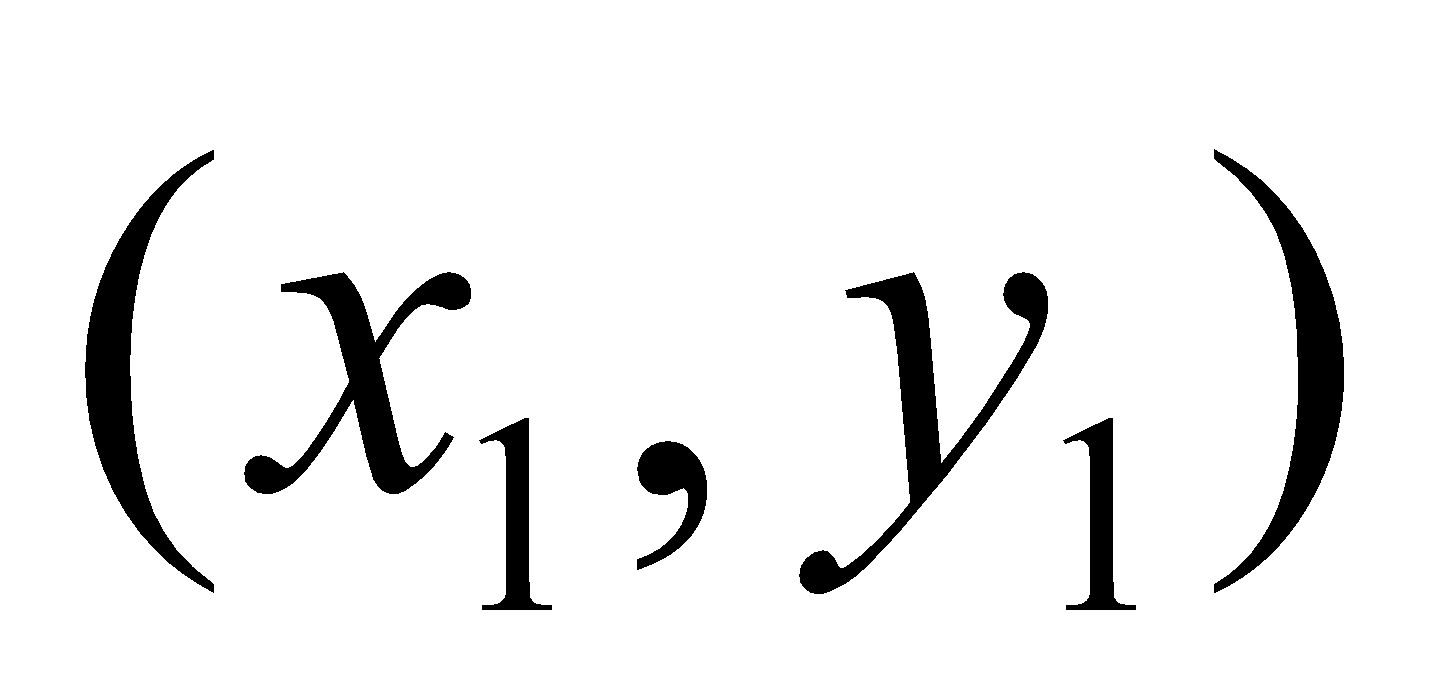to the conic 4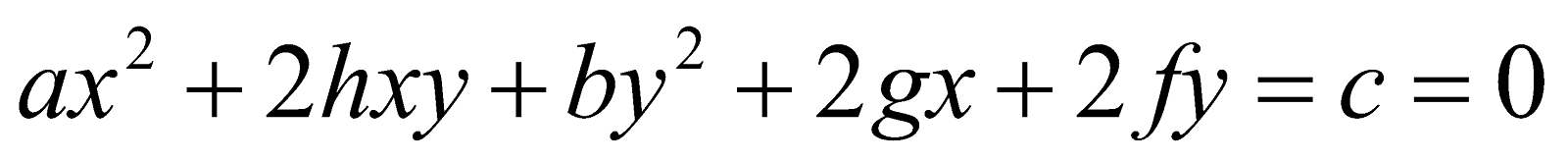Or
Show that the line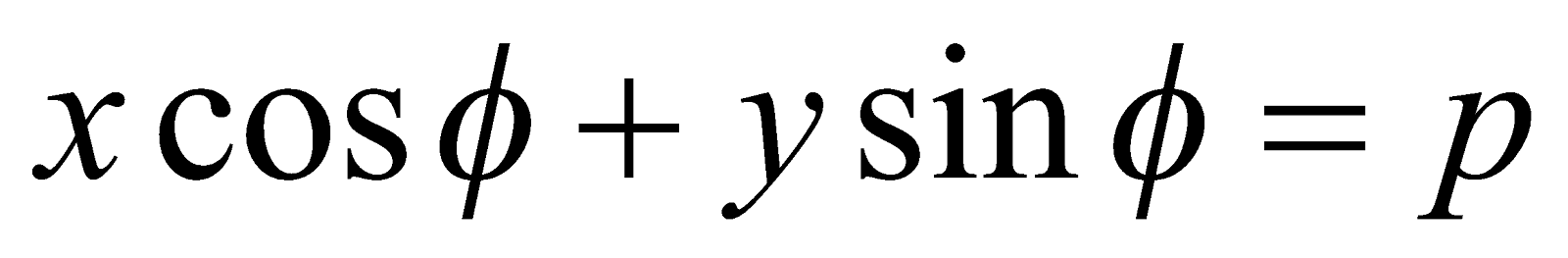will be a tangent to the conic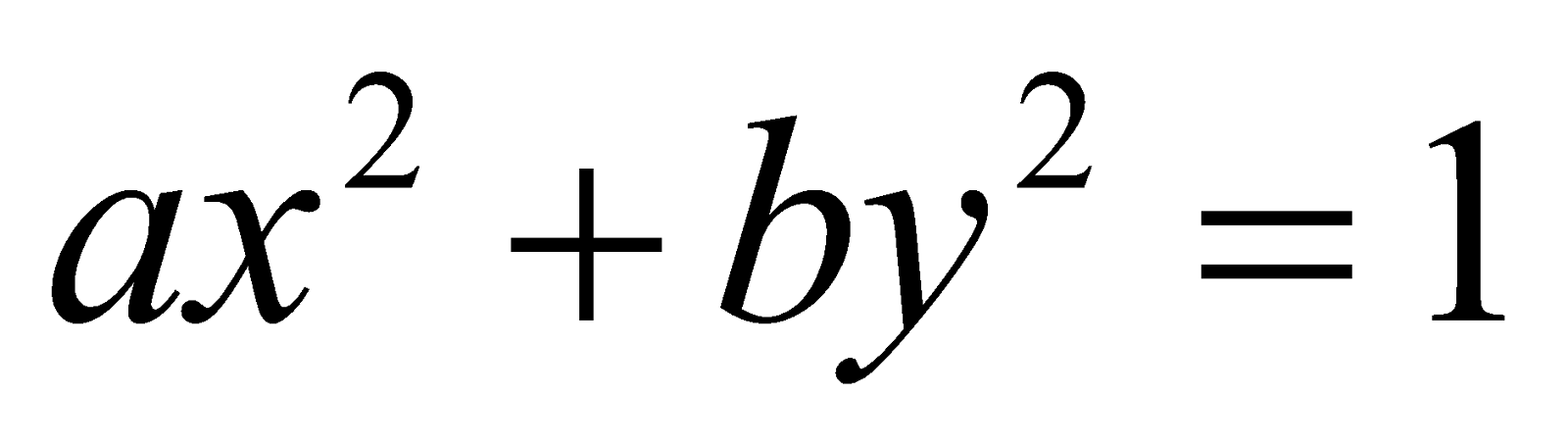if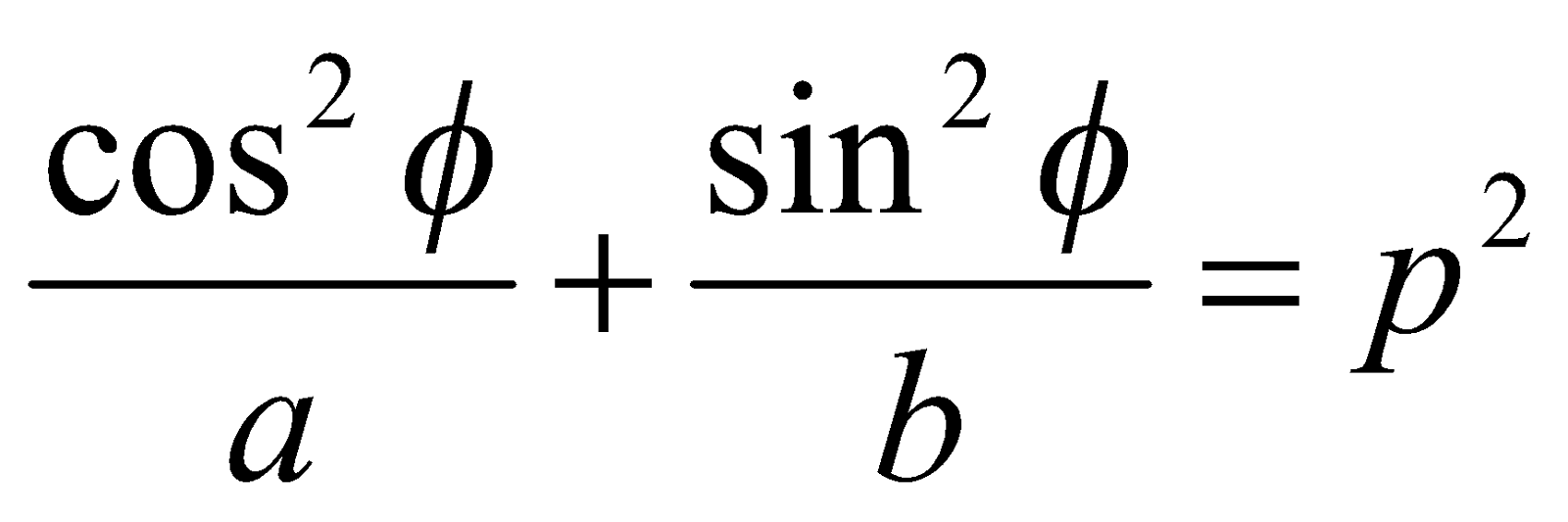(c) Define Chord of contact, diameter and conjugate diameter. 2+1=3
Or
Determine the pole of the line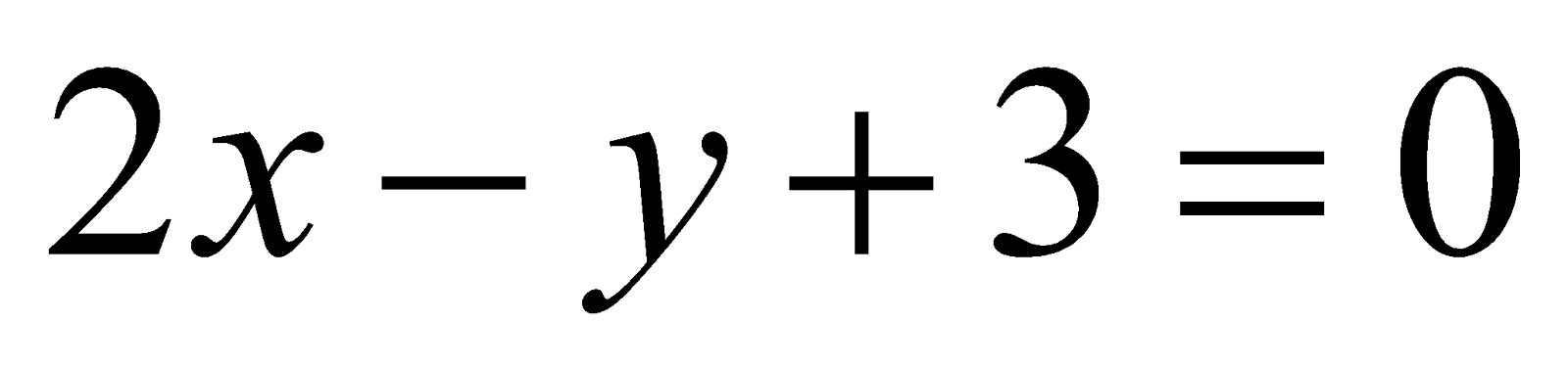with respect to the conic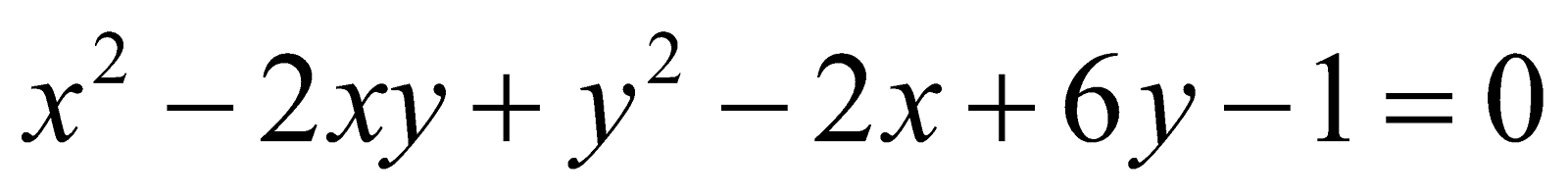.

SECTION – II
(3-Dimension)
4. (a) Write the direction cosines of any normal to the plane.
(b) Find the points of intersection of the plane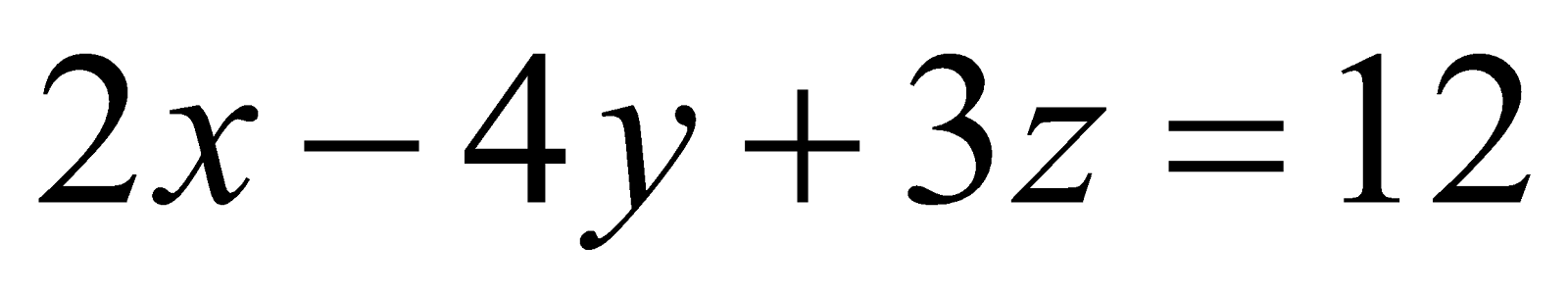with the coordinate axes.
(c) What is the equation of the plane parallel to the plane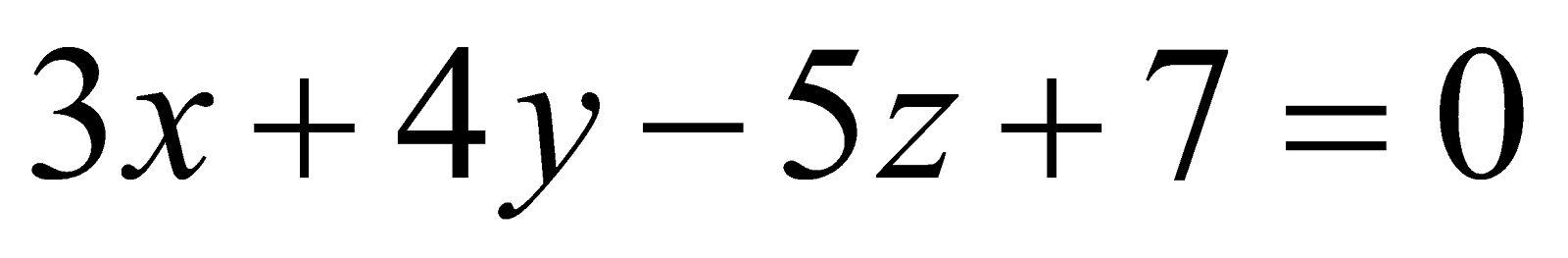and passing through the origin?
(d) Find the equation of the plane passing through the point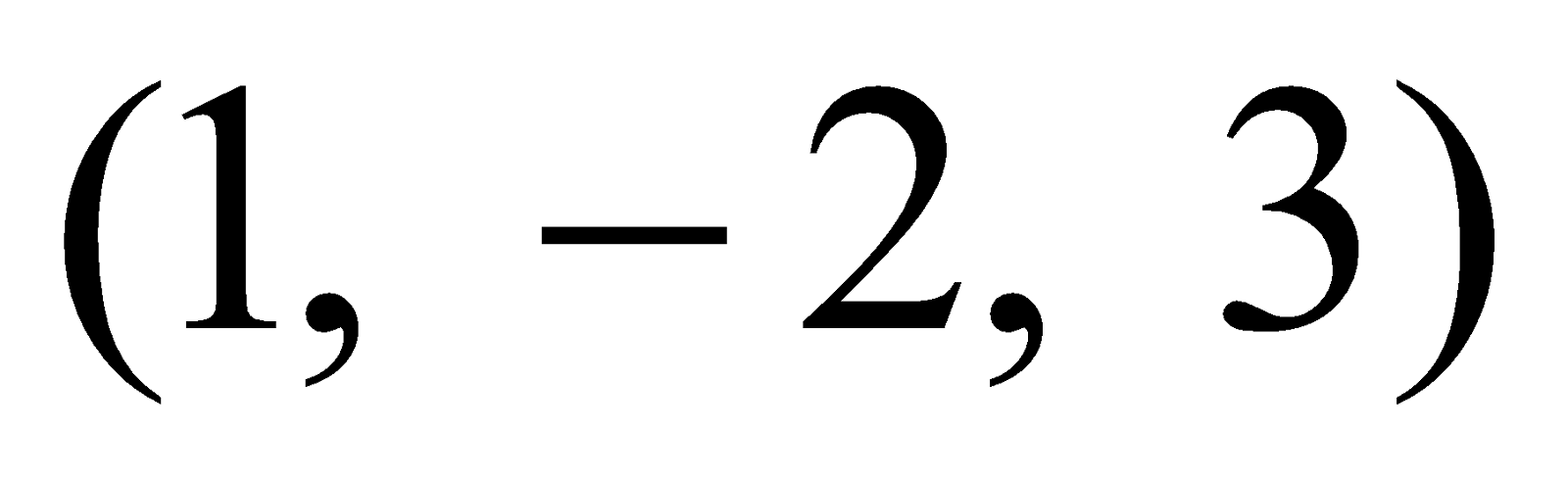and perpendicular to each of the planes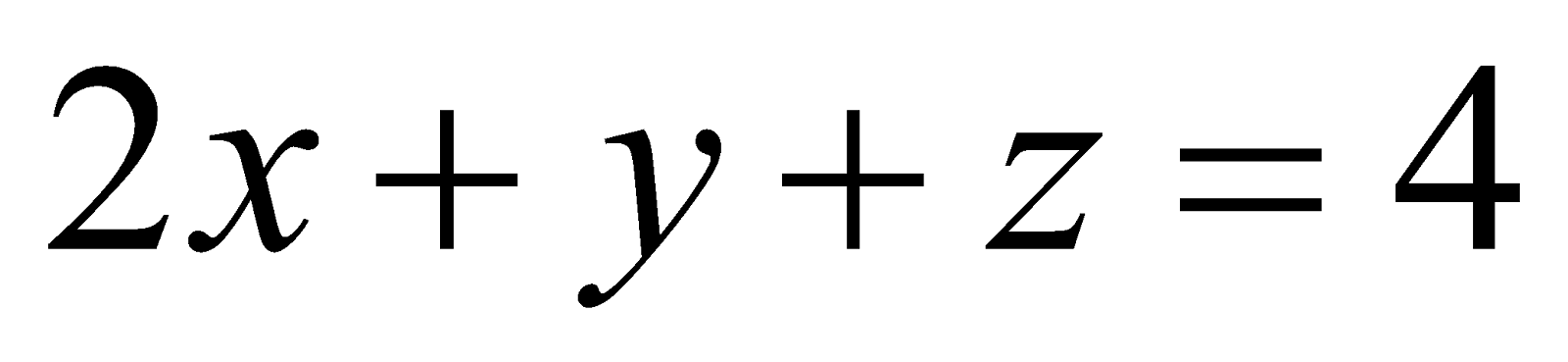and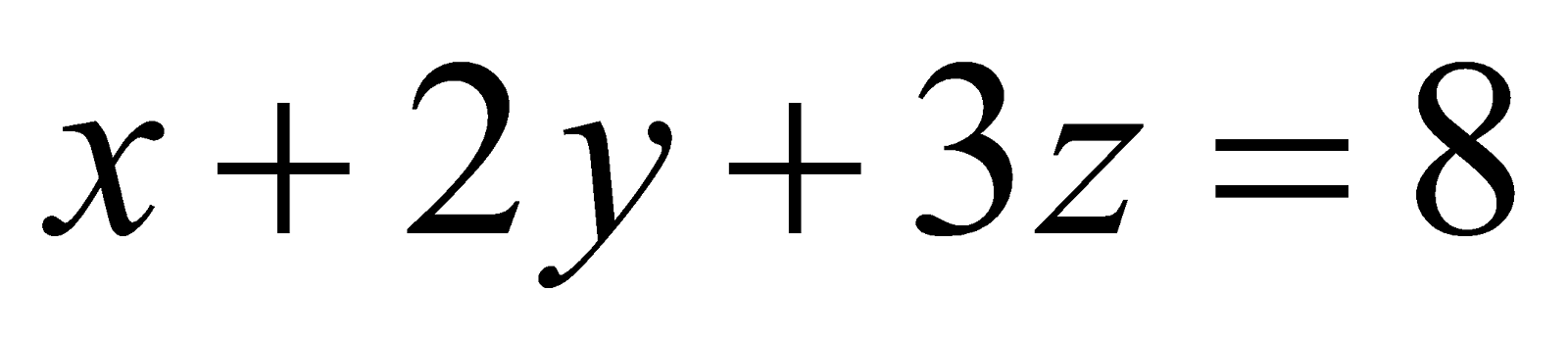. 3
Or
Find the locus of the point whose distance from the origin is three times its distance from the plane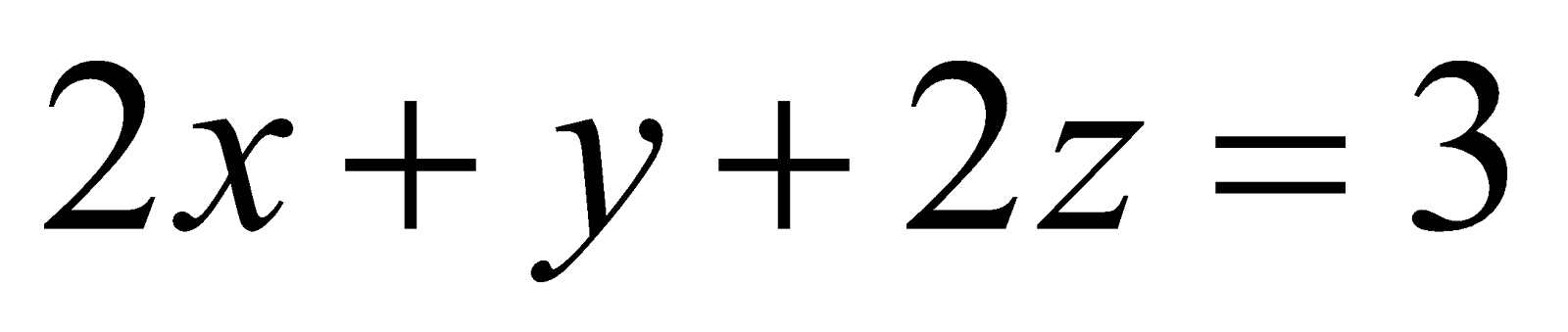.
(e) Find the bisector of that angle between the planes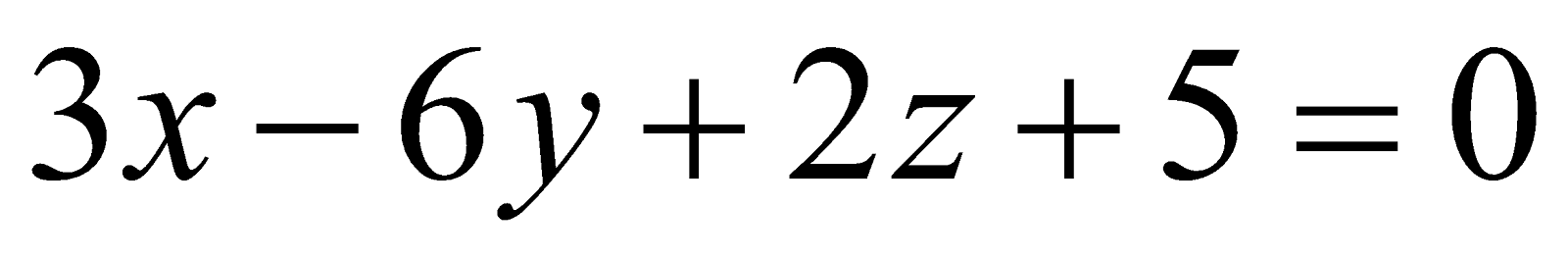and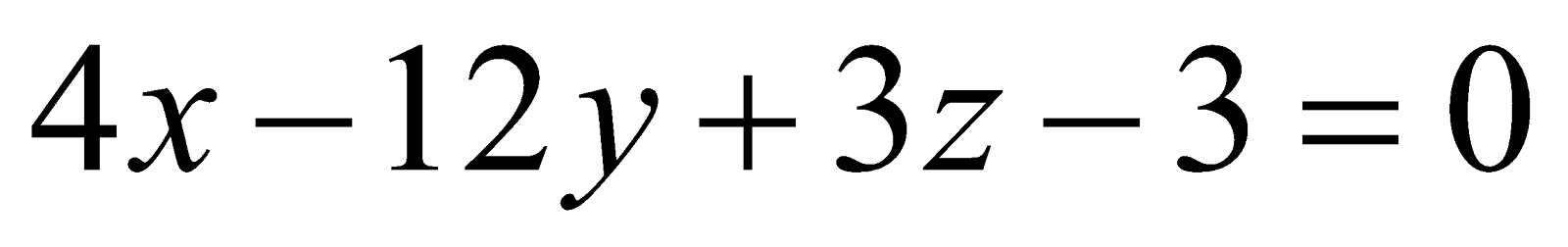which contains the origin. 4
Or
Find the condition of representing two planes by the equation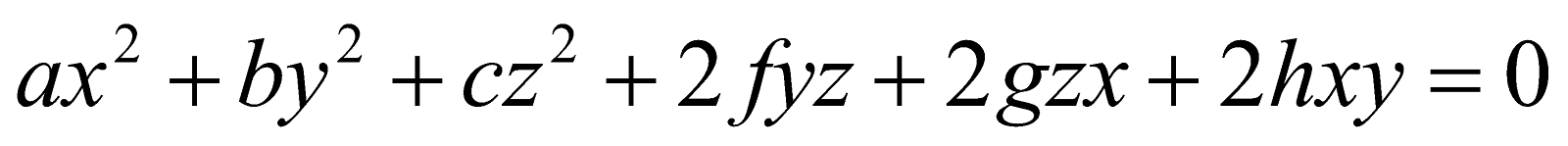.
5. (a) Show that the two lines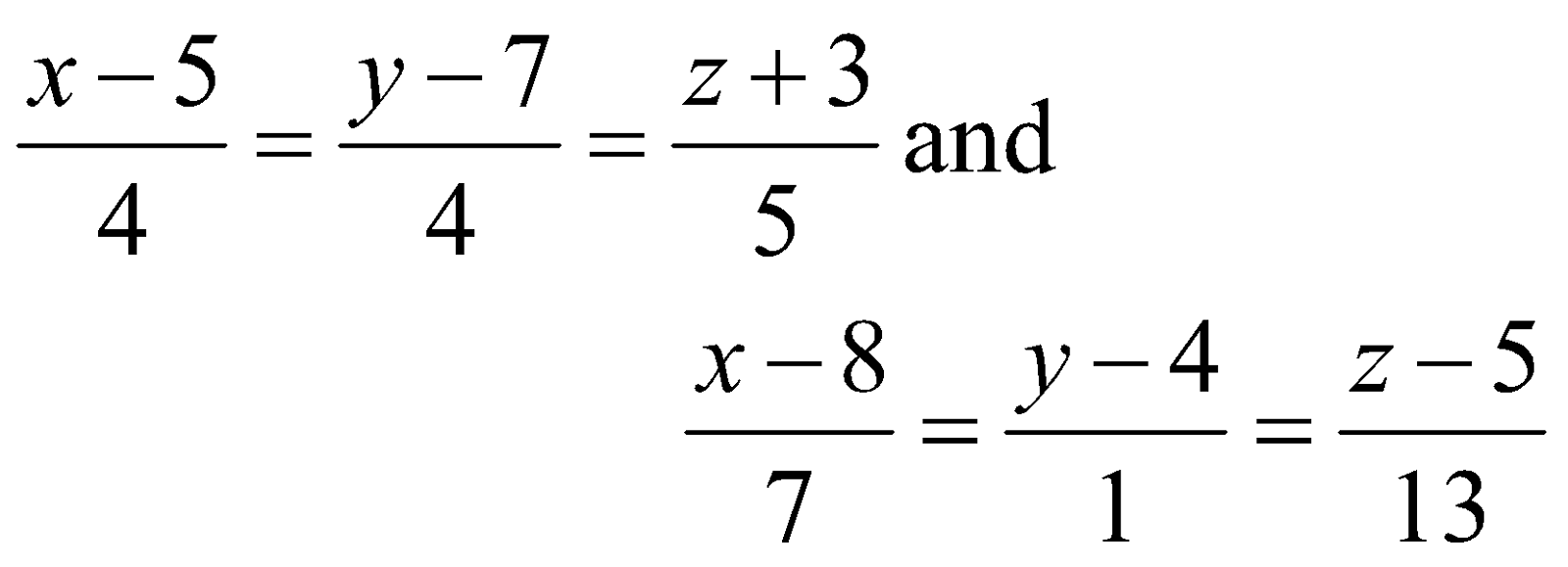are coplanar. Find the equation of the plane through the two lines.
Or
Prove that the plane through the line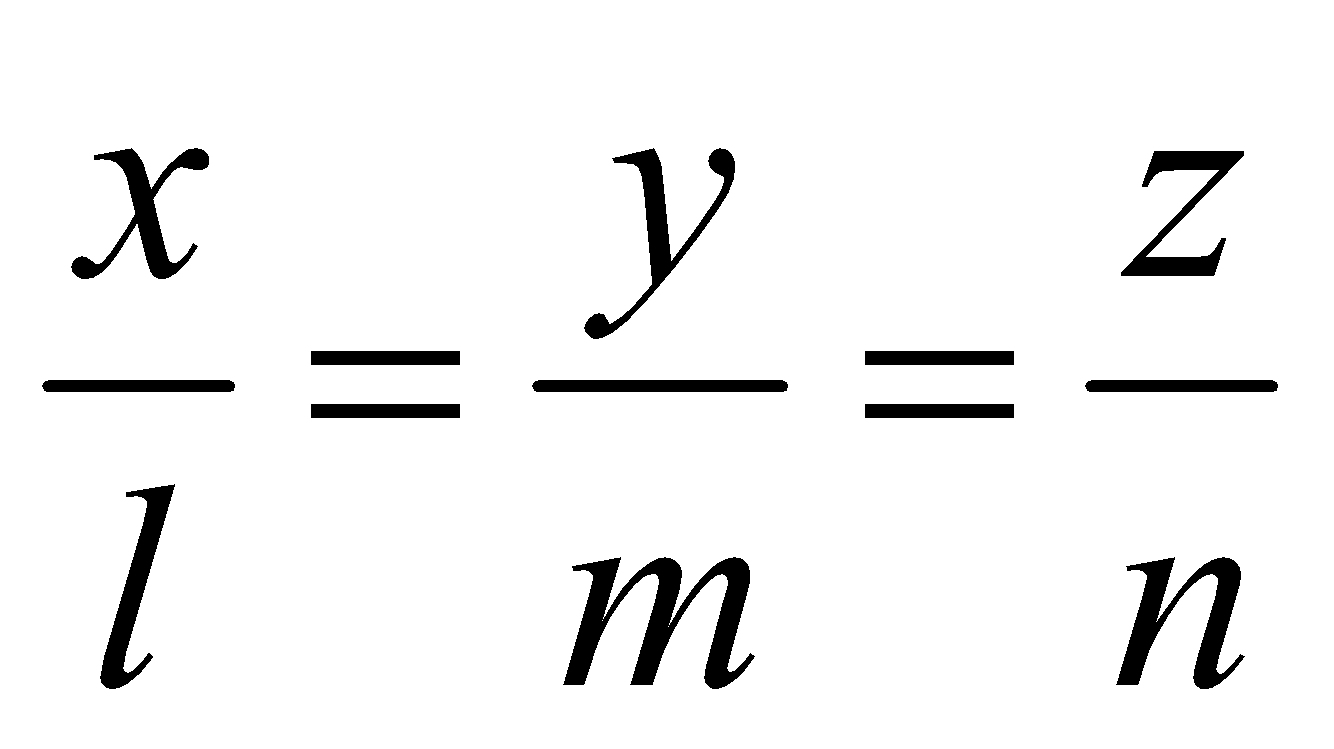and perpendicular to the plane through the lines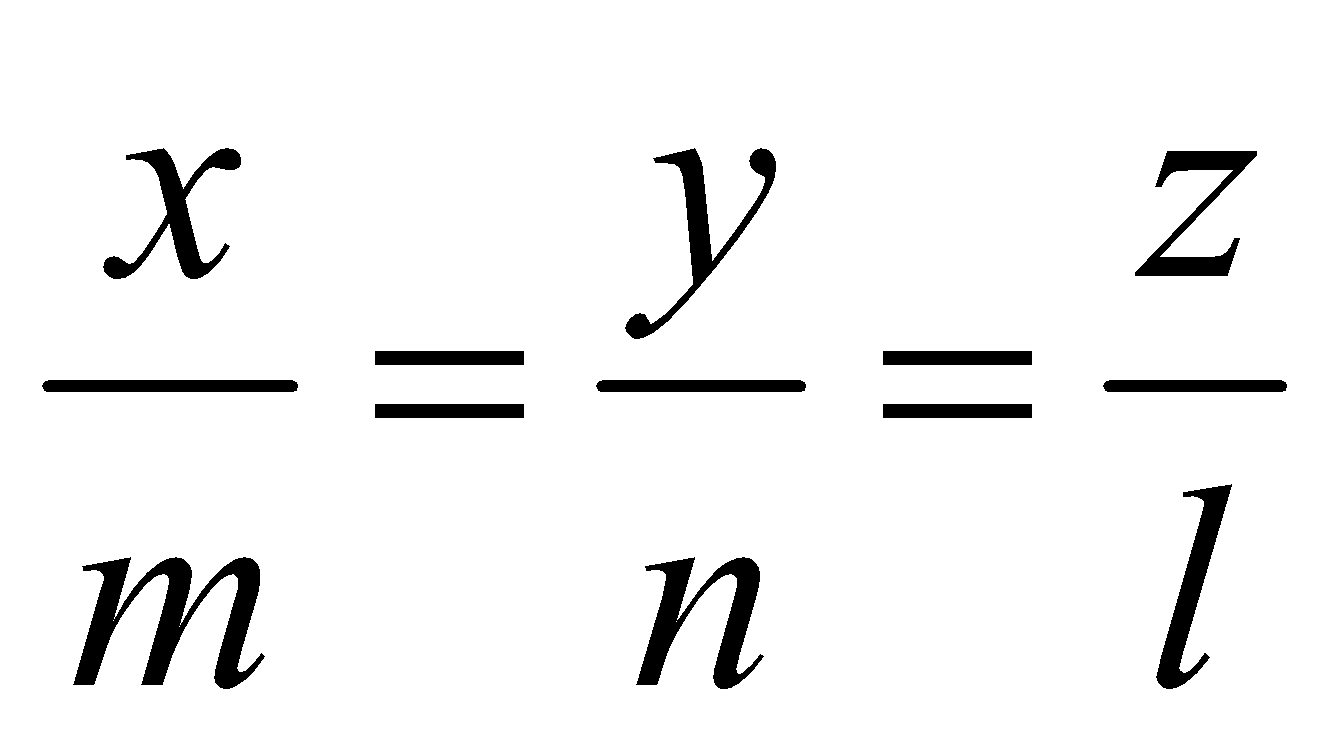and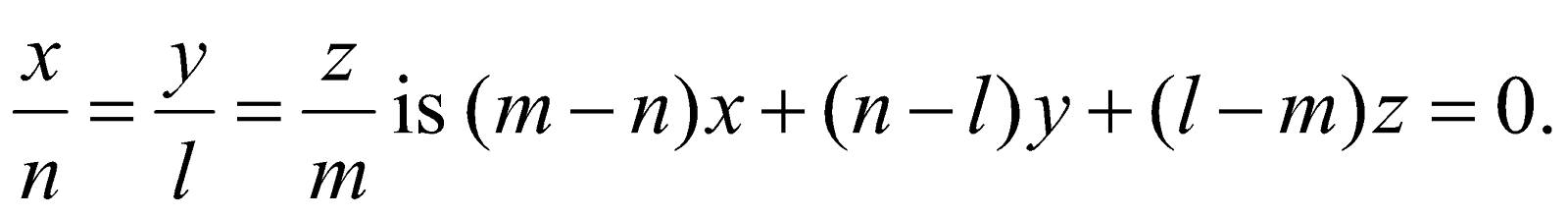(b) Determine the shortest distance between the lines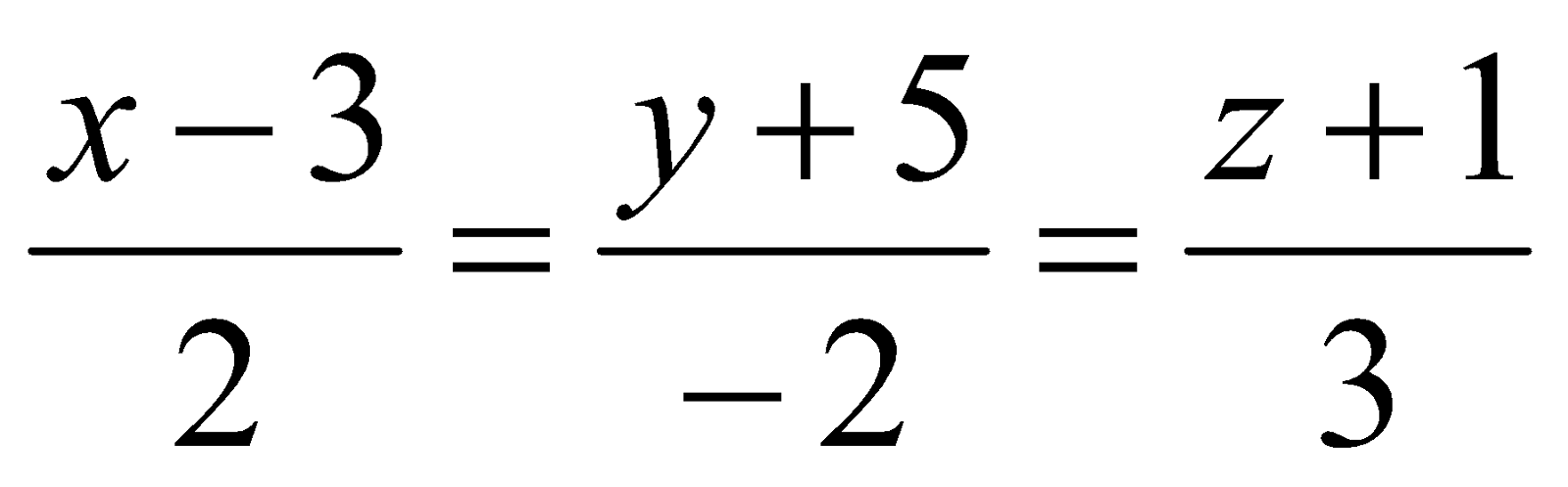and theaxis. 4
Or
Find the equation of the line of shortest distance between the lines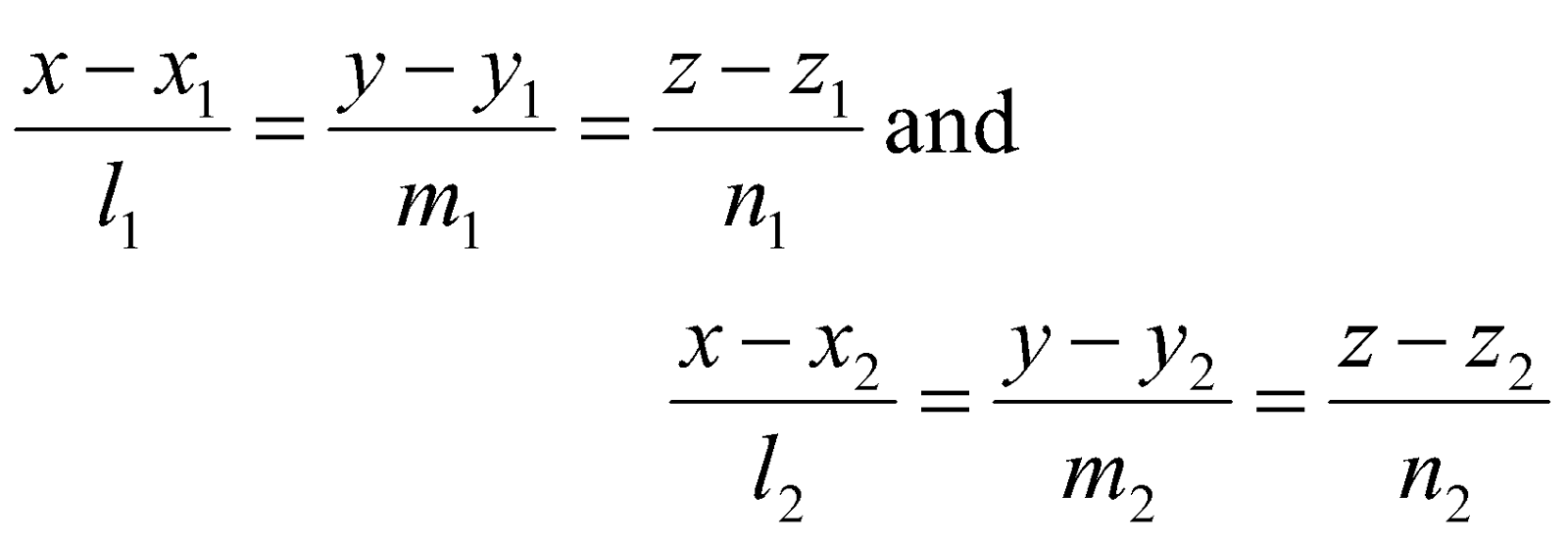GROUP – B
(Analysis – I)
6. (a) If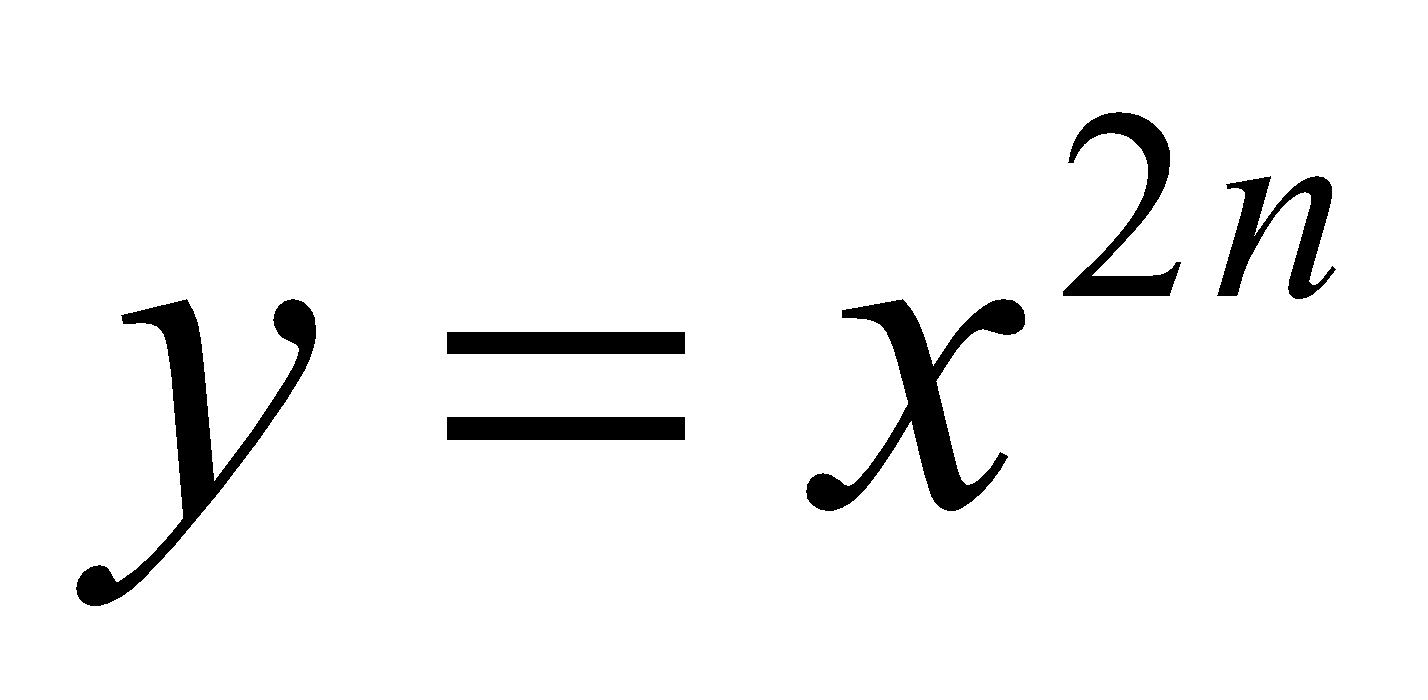, where n is a positive integer, show that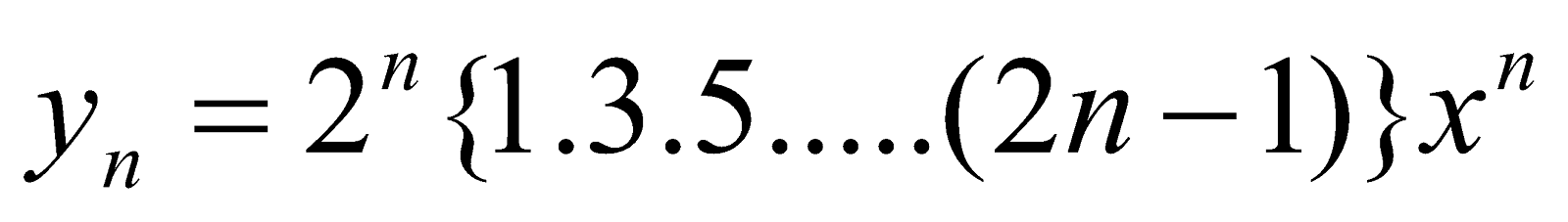. 2
(b) Find the radius of curvature of the parabola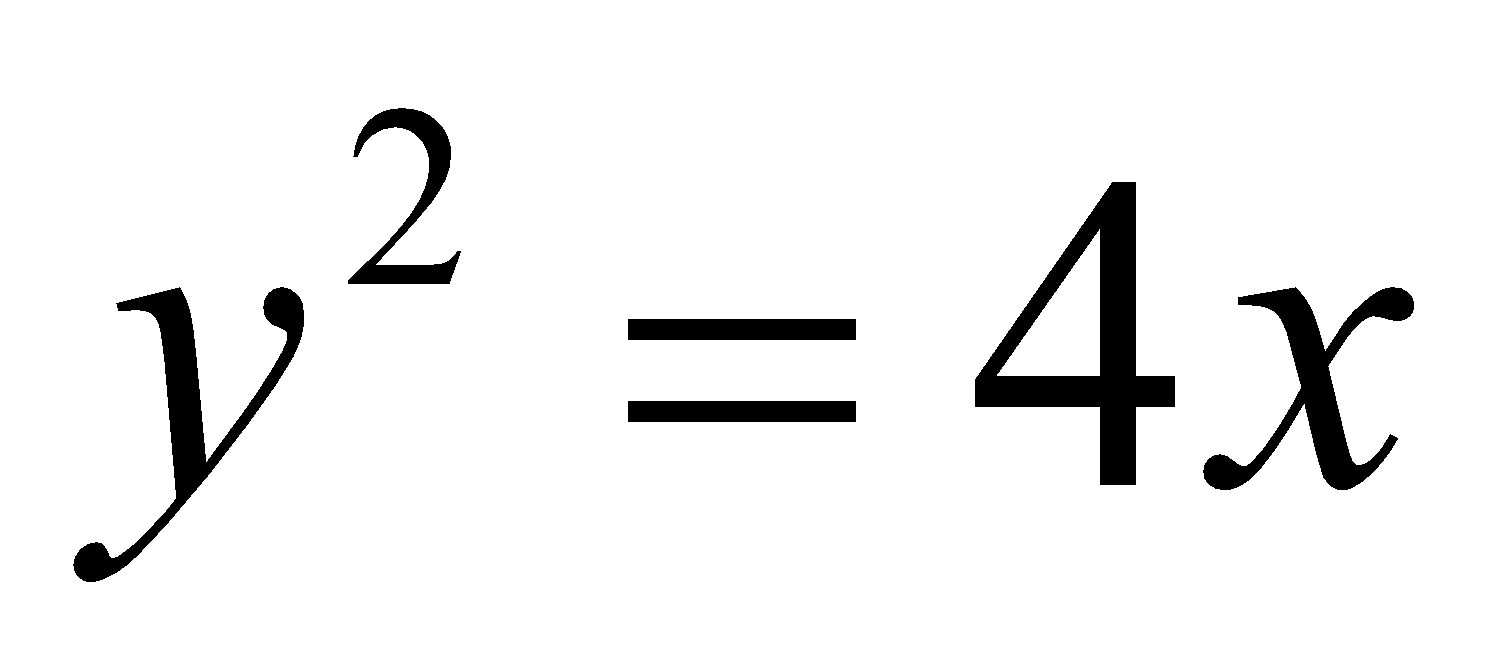at the vertex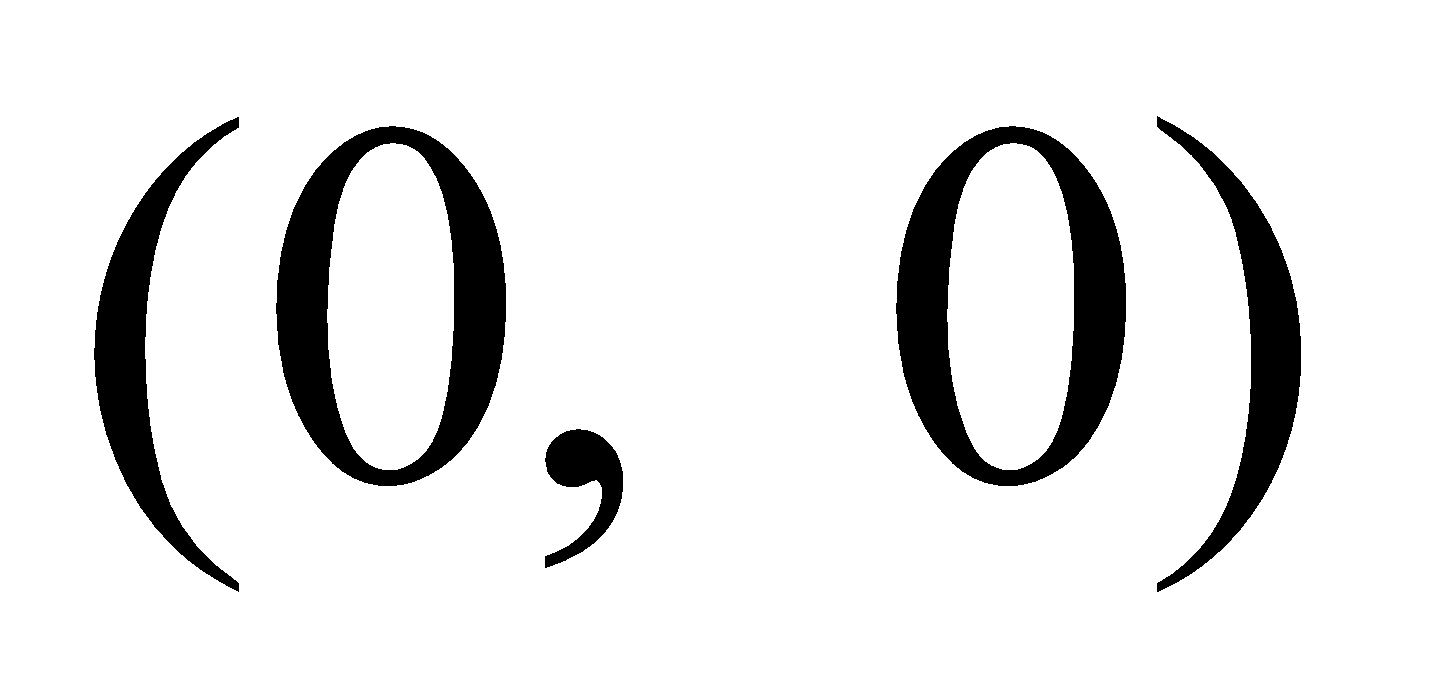. 2
(c) Show that in any curve 2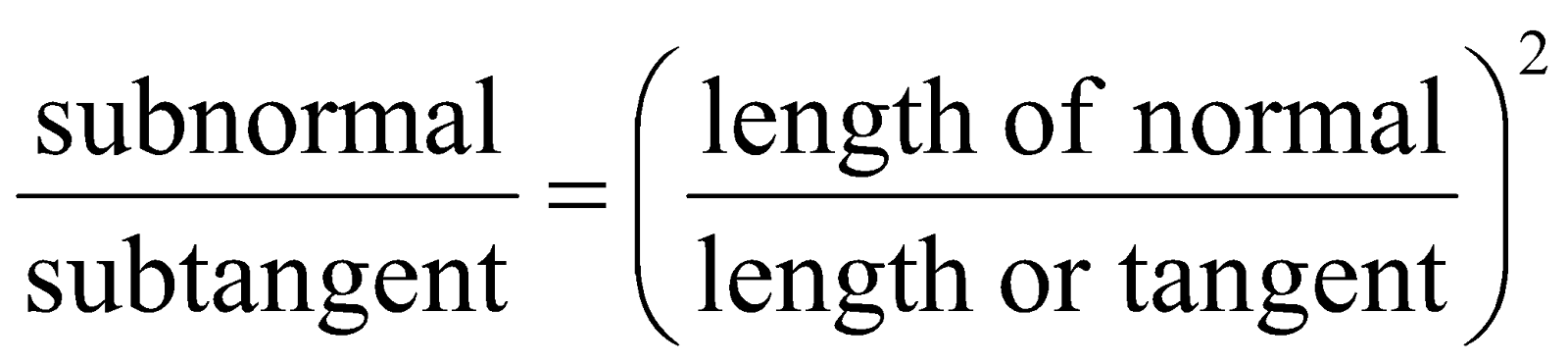(d) If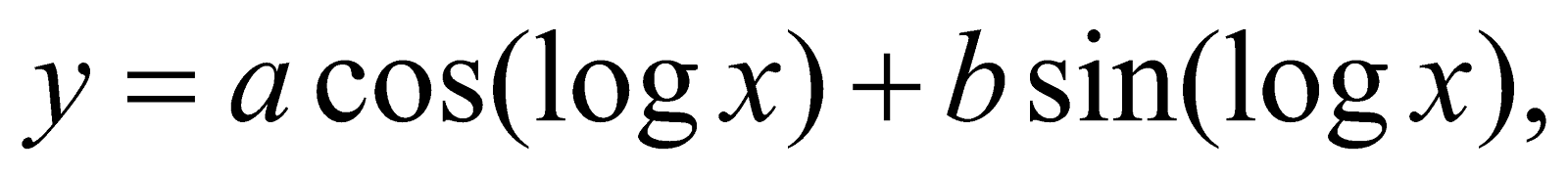show that. 4
Or
Evaluate: 2+2=4
1.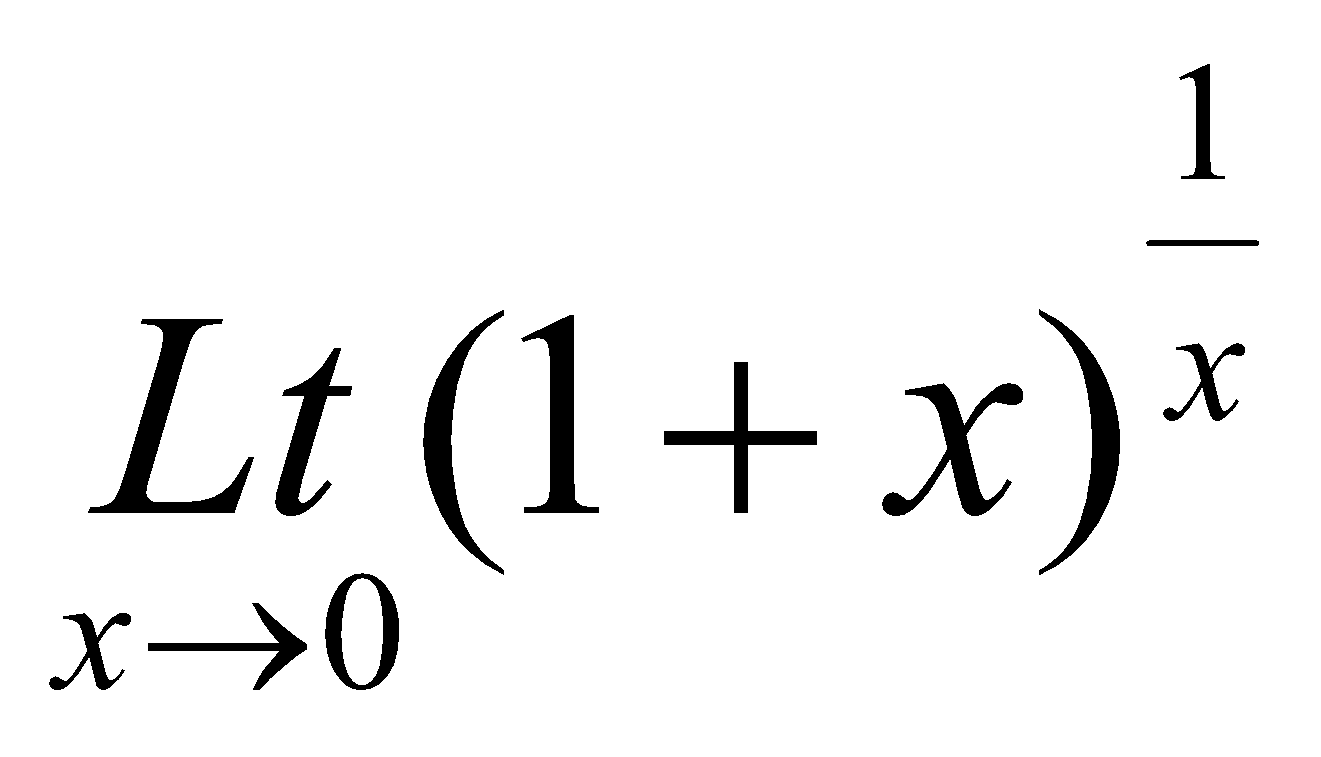2.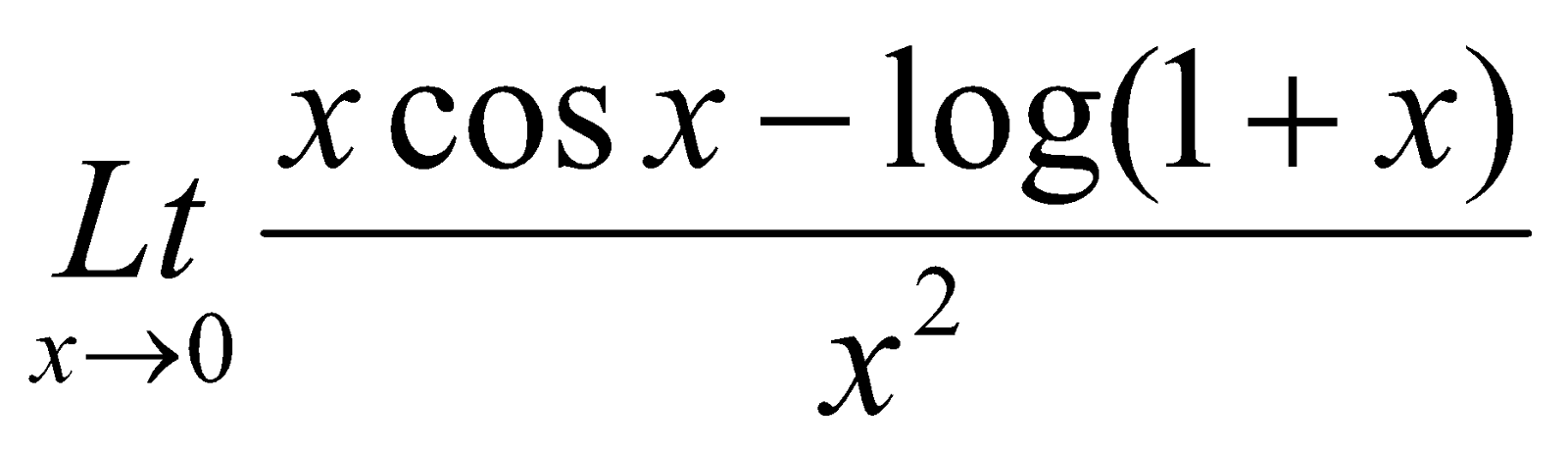7. (a) State Lagrange’s mean value theorem. 1
(b) Find the value of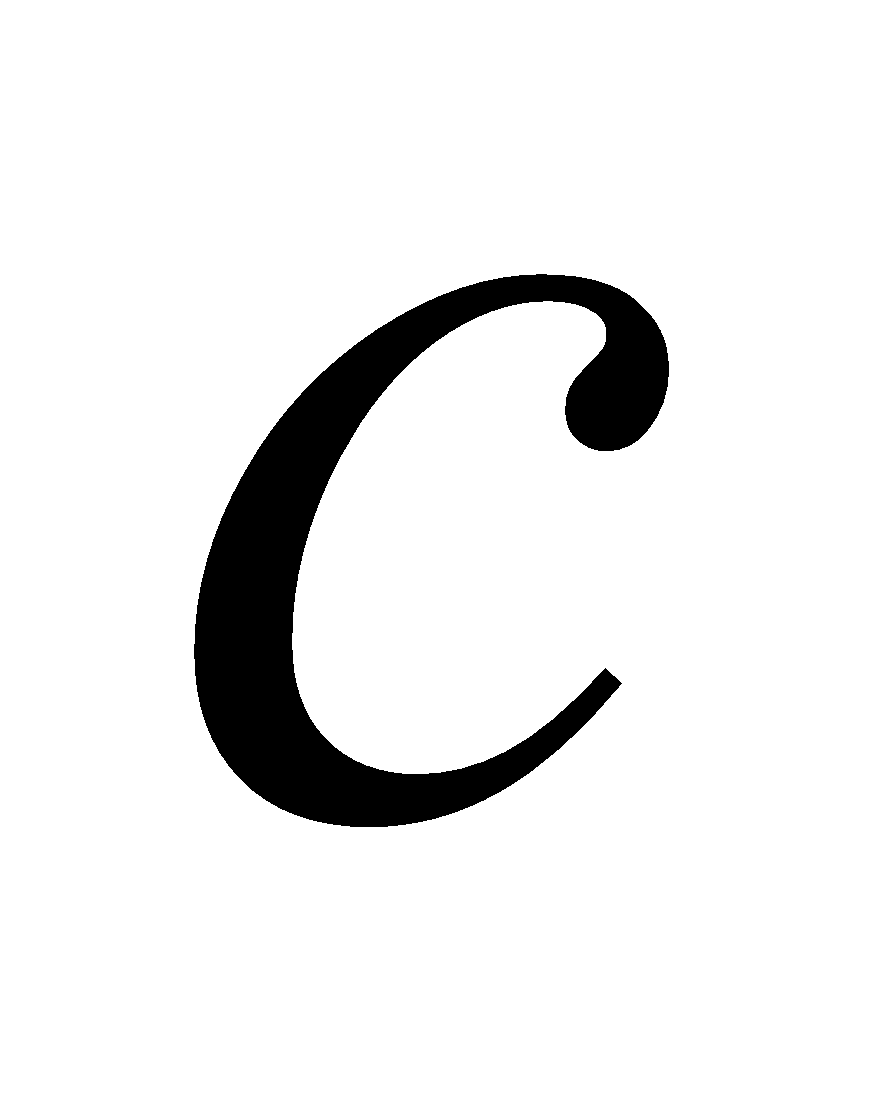in Rolle’s Theorem where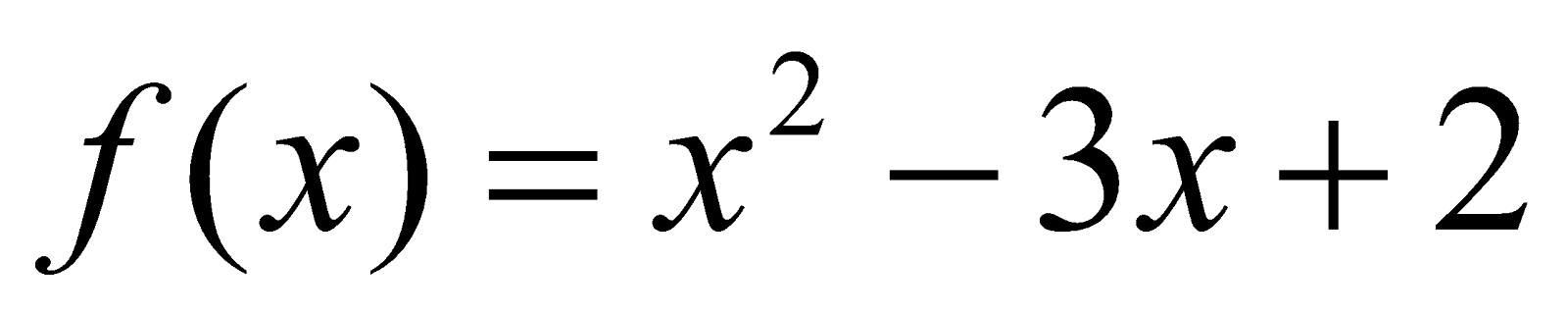in2
(c) In the mean value theorem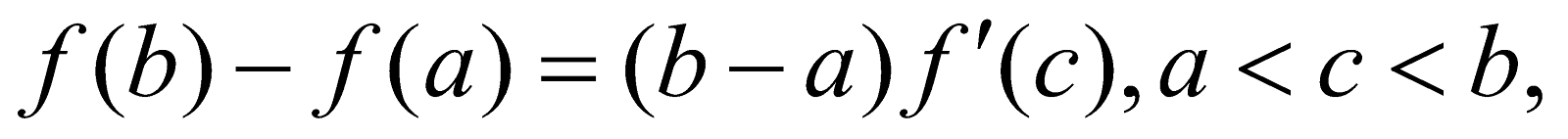find c if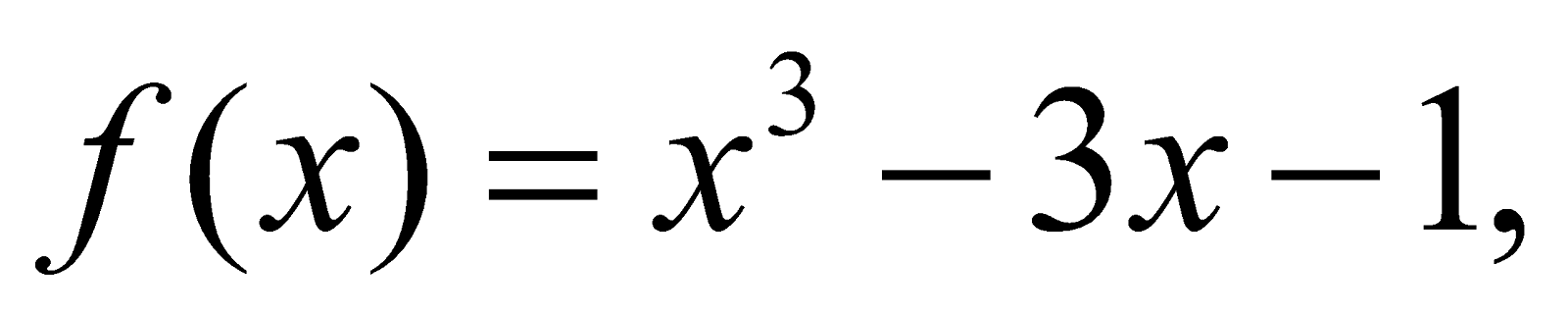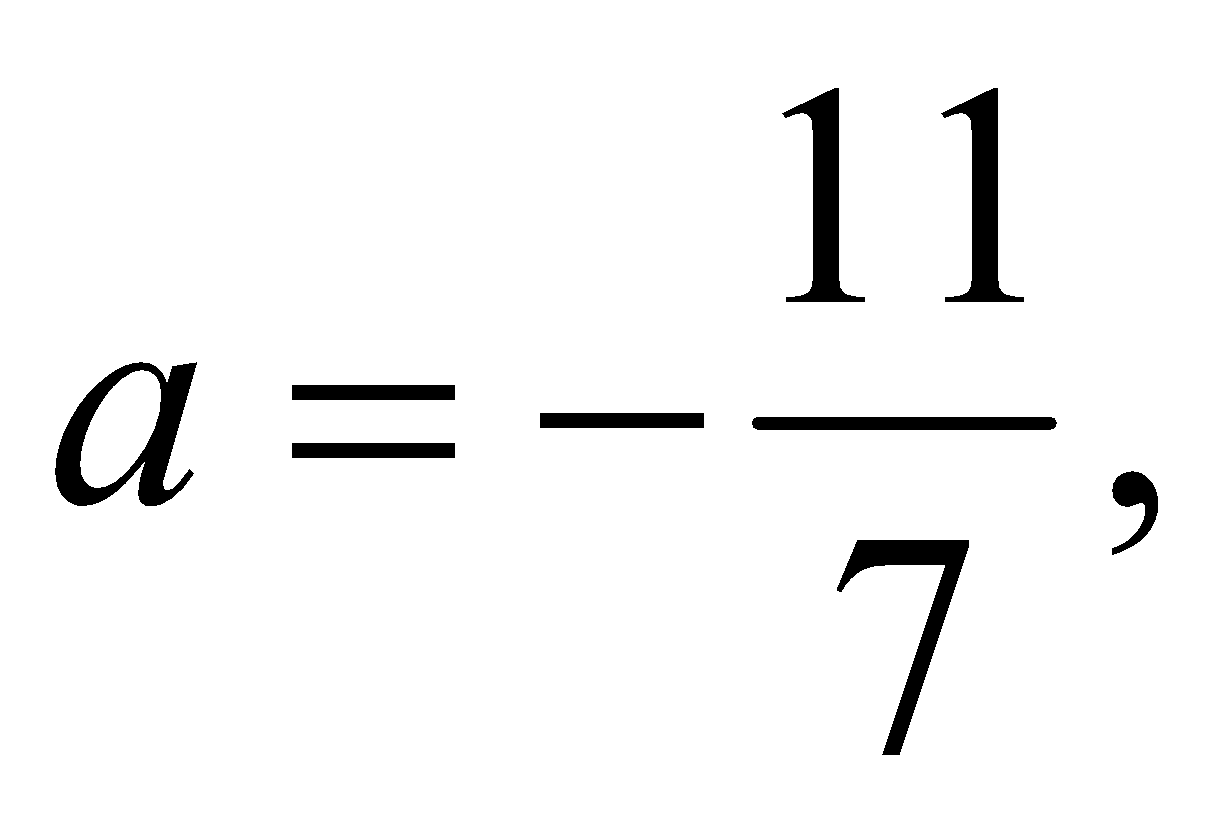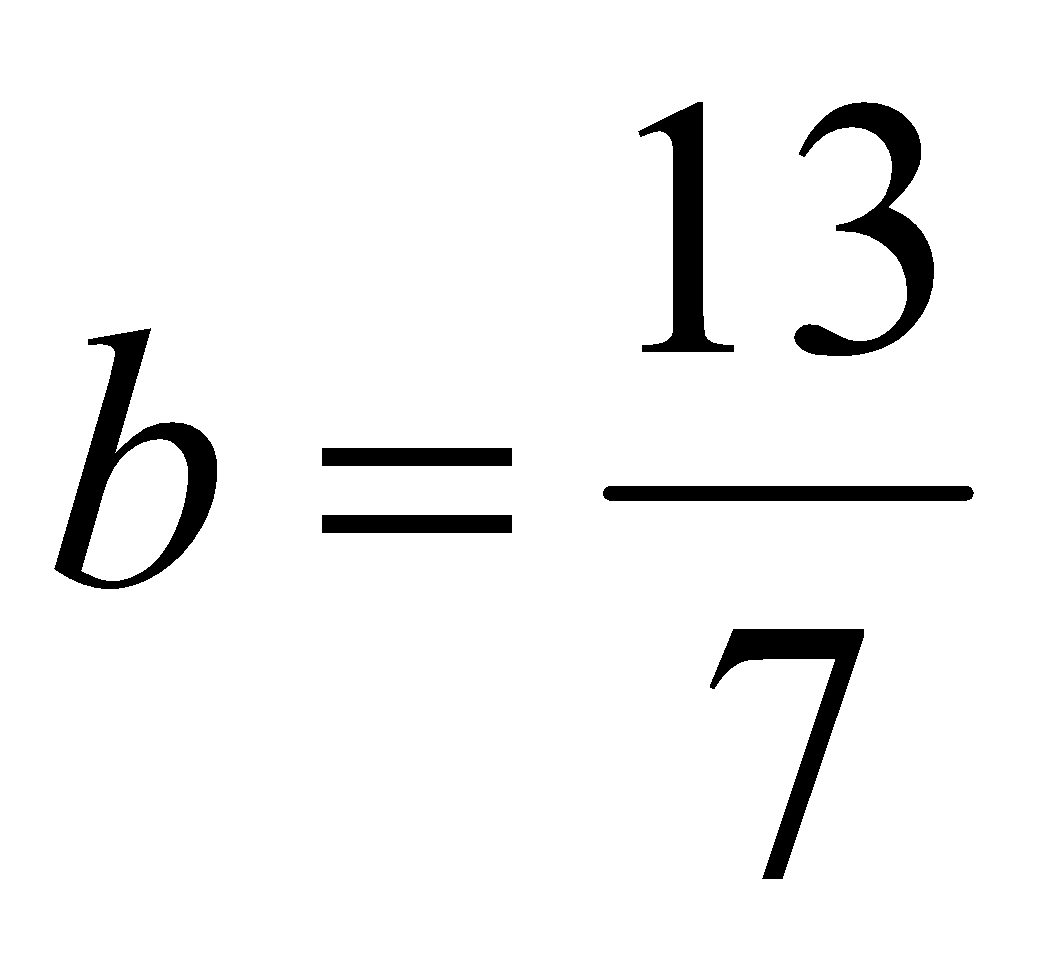and give a geometrical interpretation of the result. 3+1=4
Or
If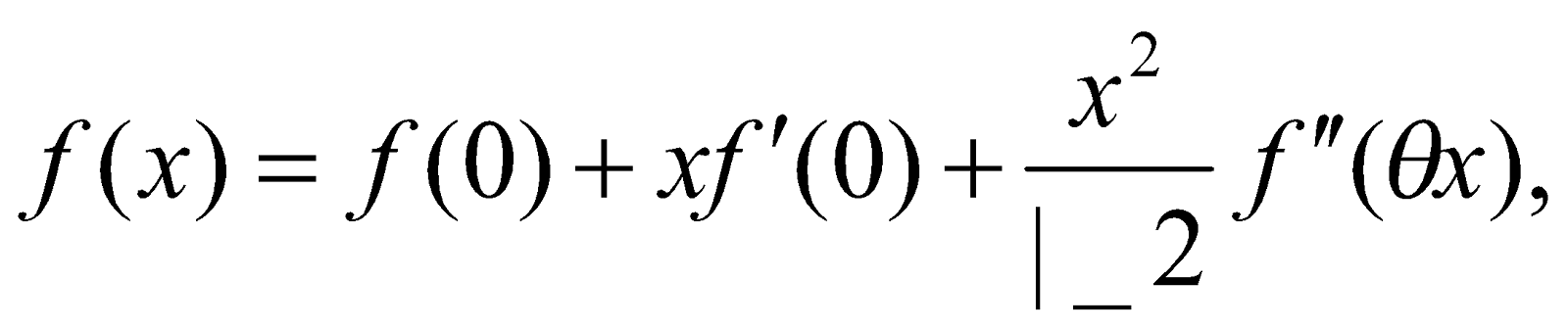find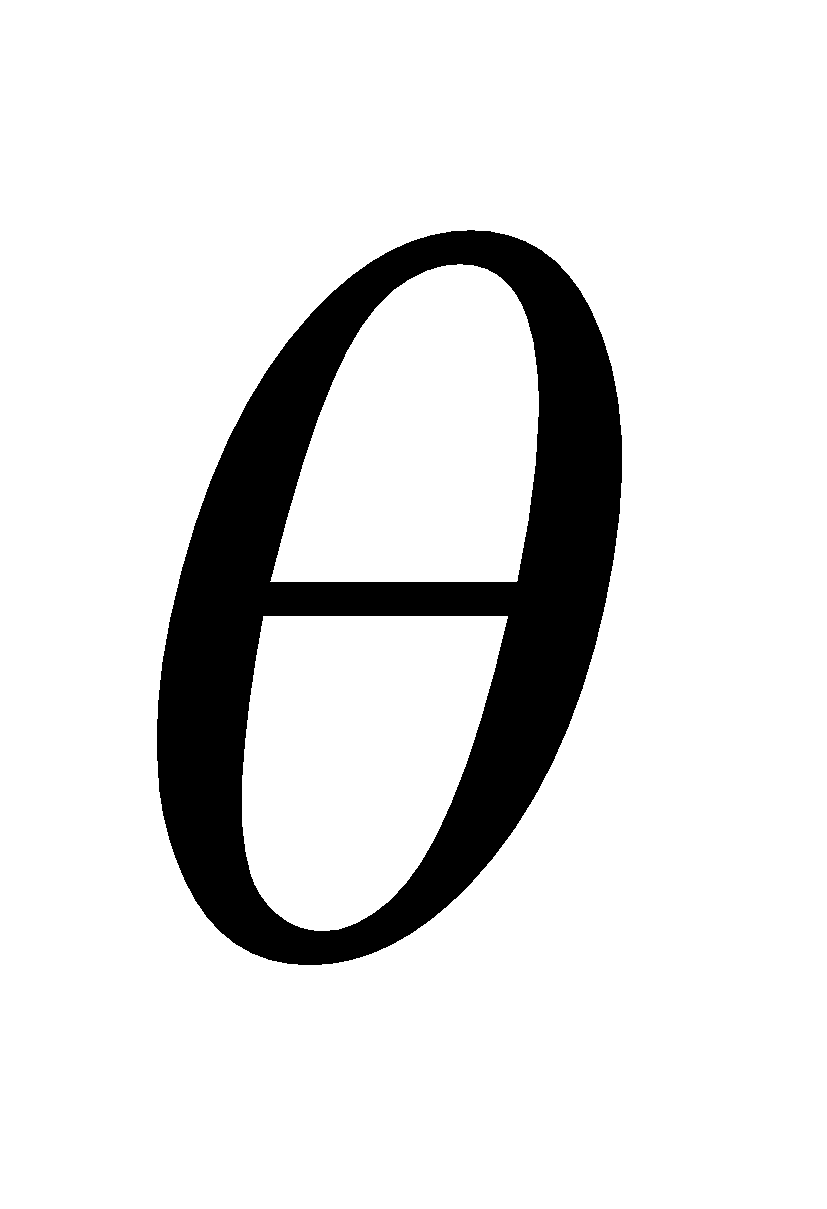where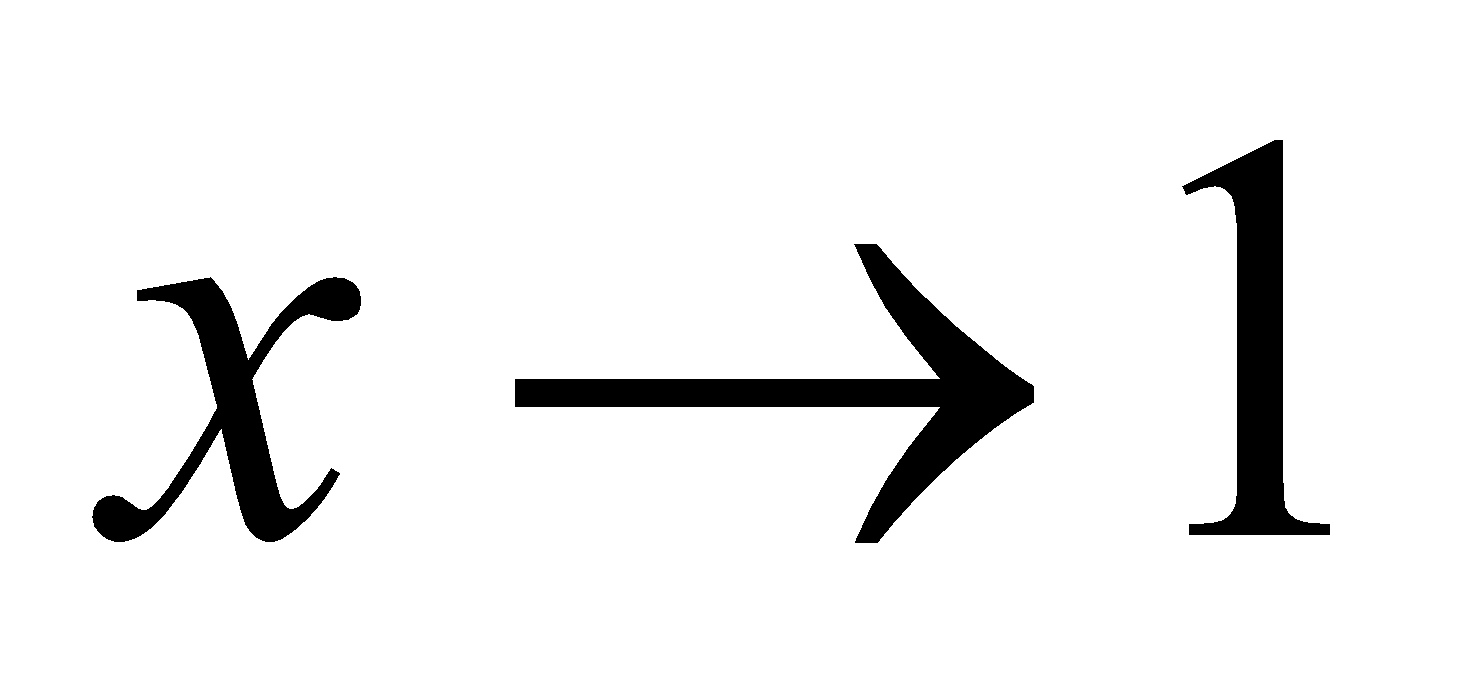and where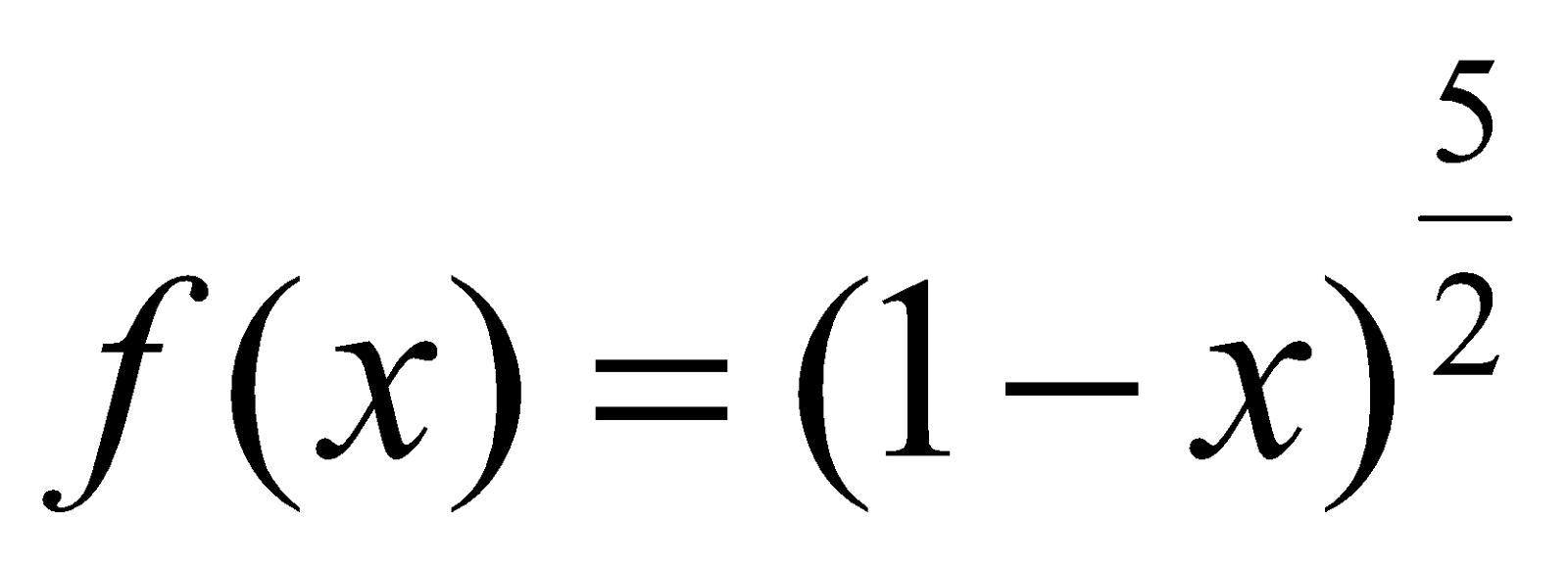. 4
(d) Show that the function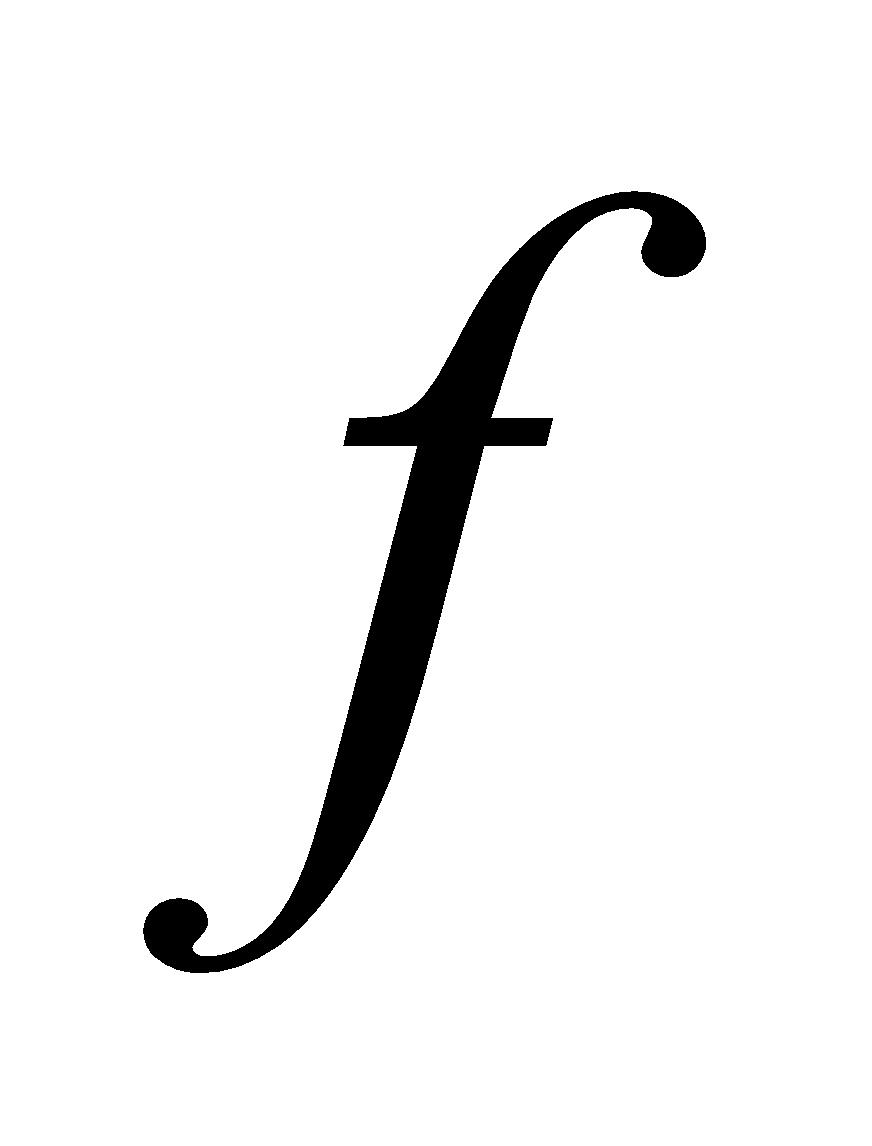denied by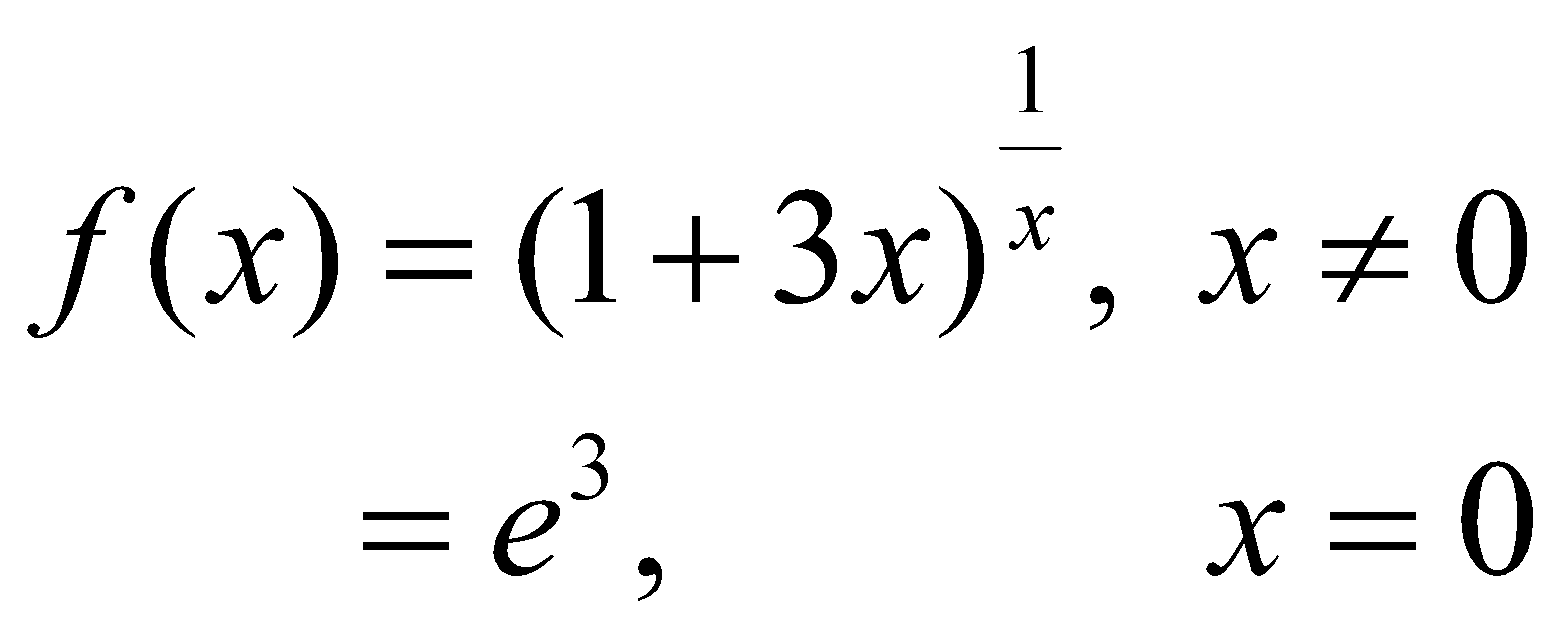Is continuous at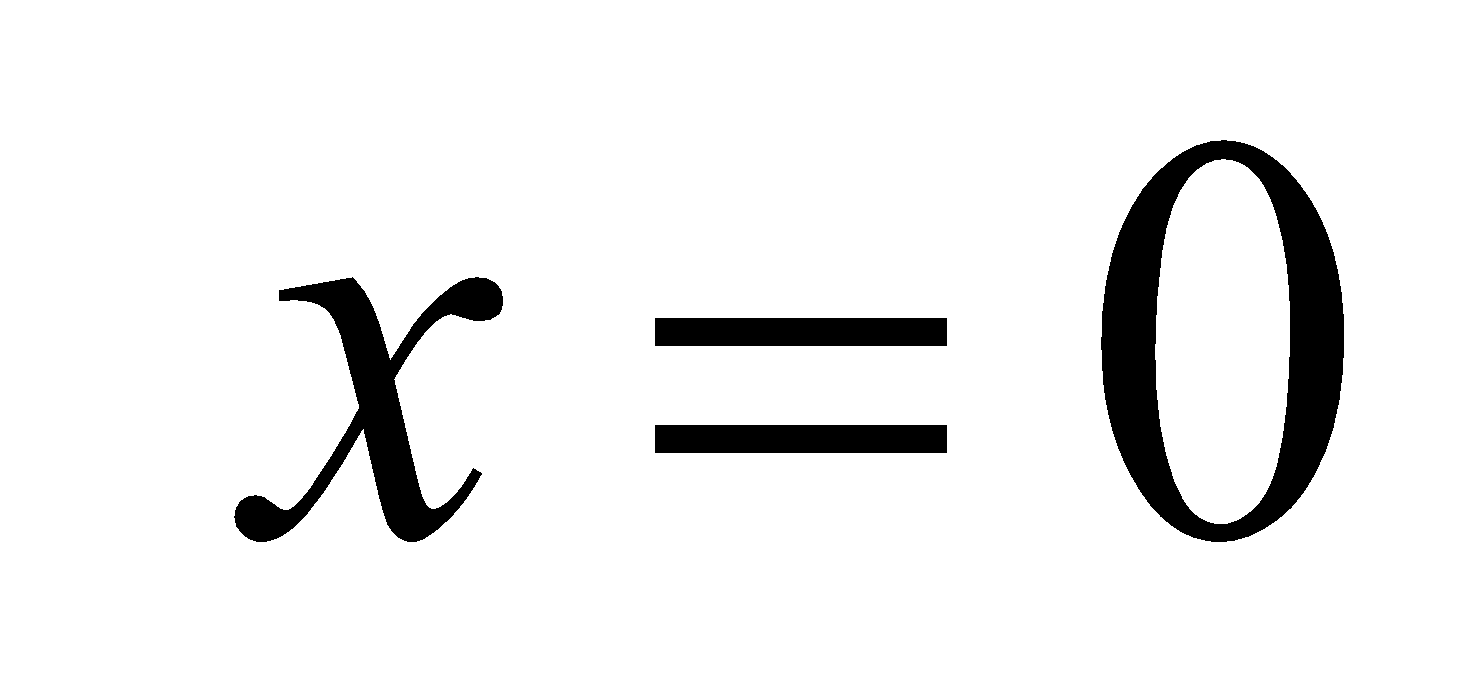8. (a) State and prove Euler’s theorem on homogeneous function of two variables. 3
Or
If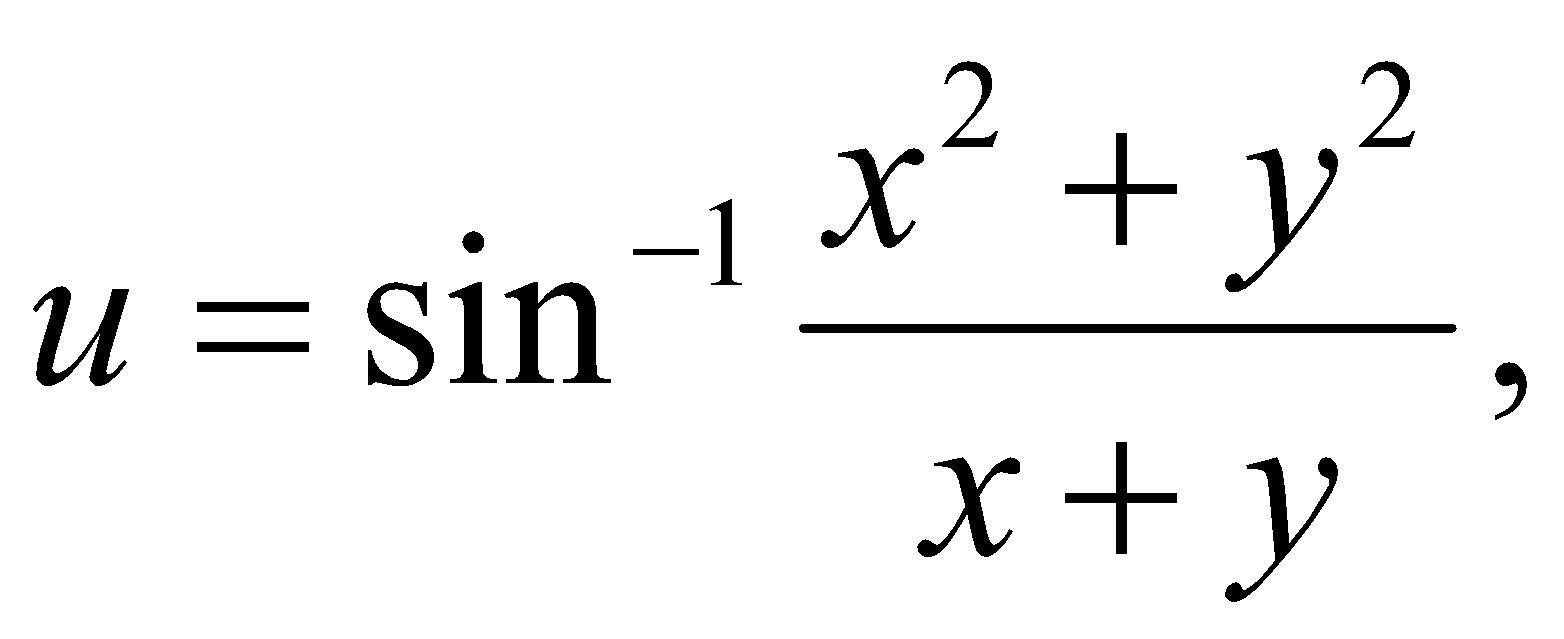then show that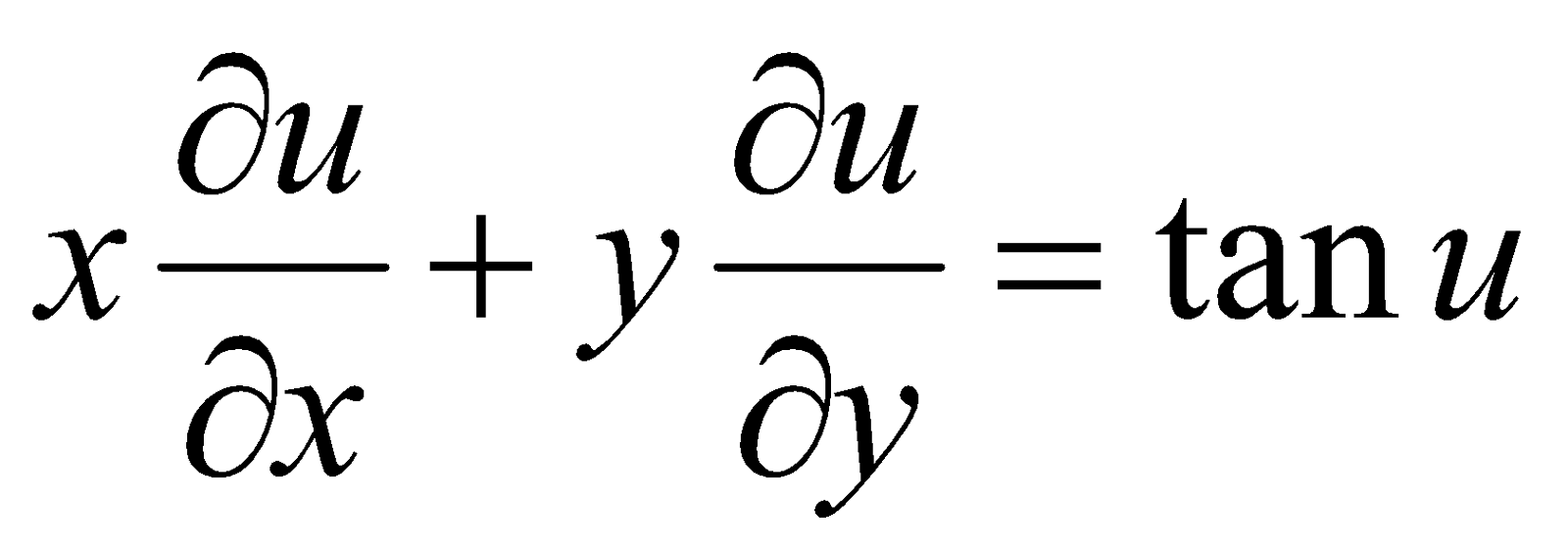(b) Show thatif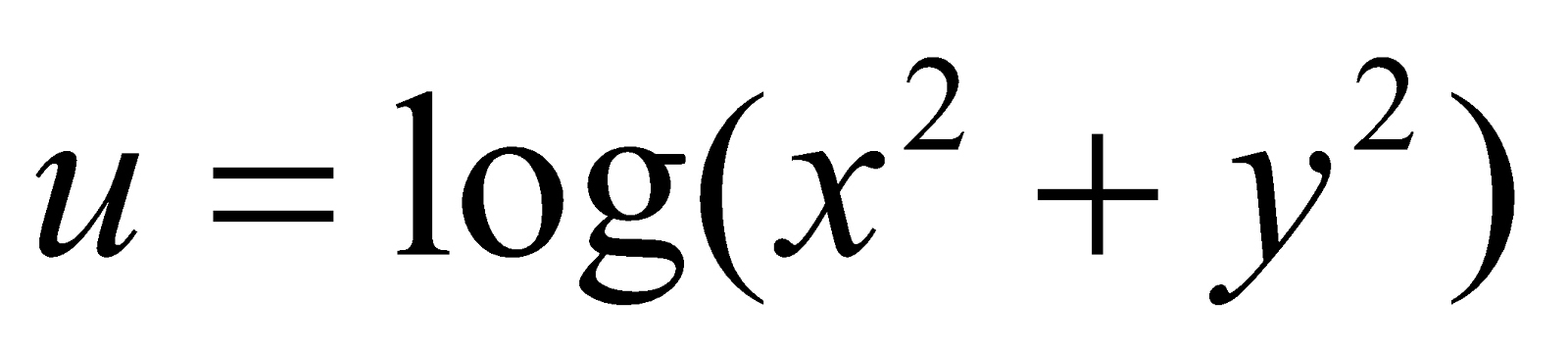. 2
9. (a) Prove that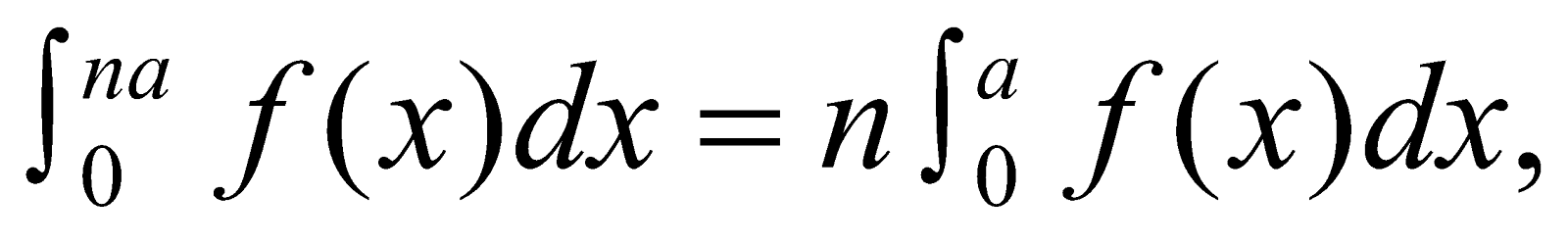if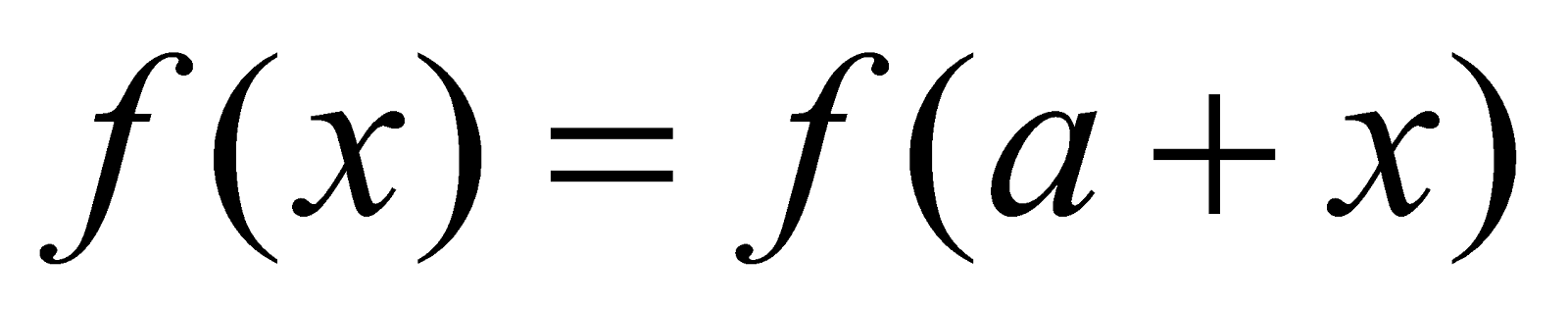3
Or
Show that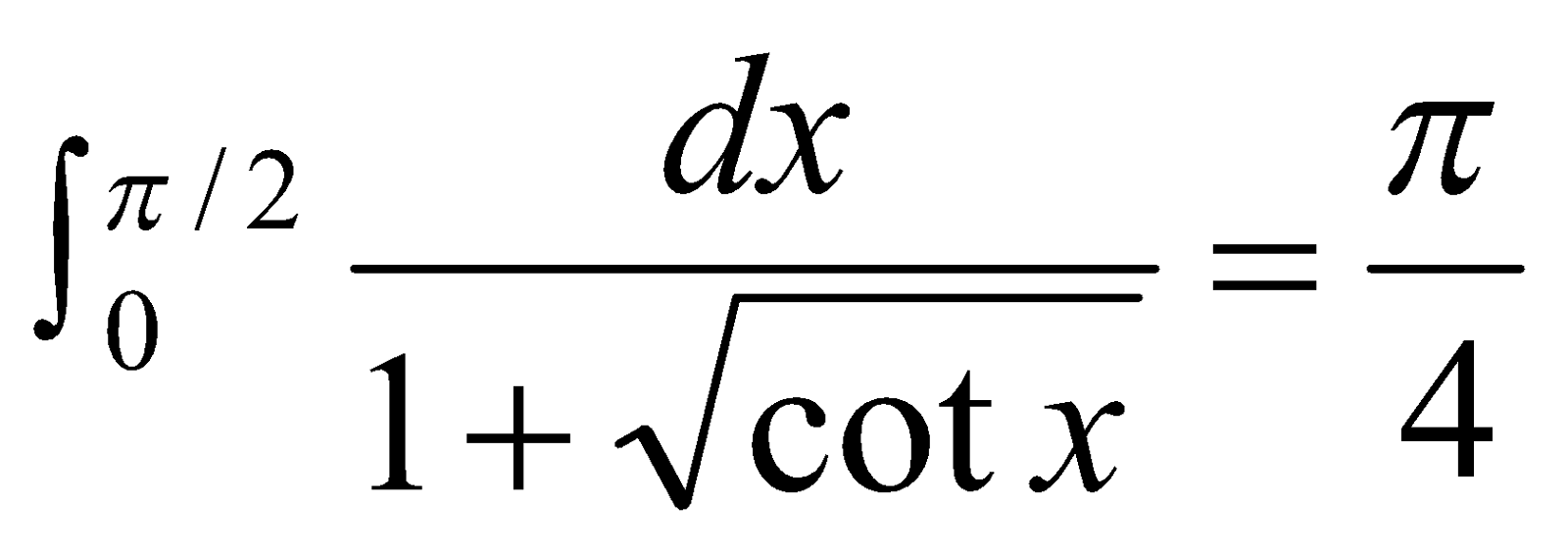(b) If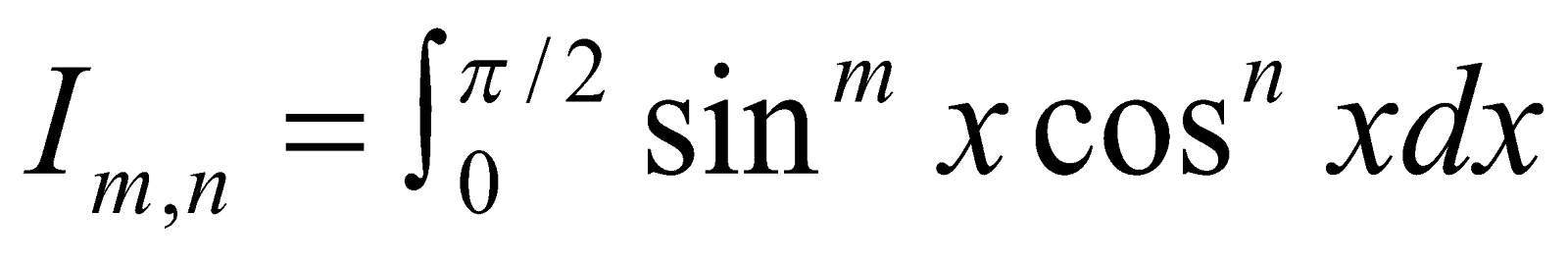(m, n being positive integers), then deduce that 5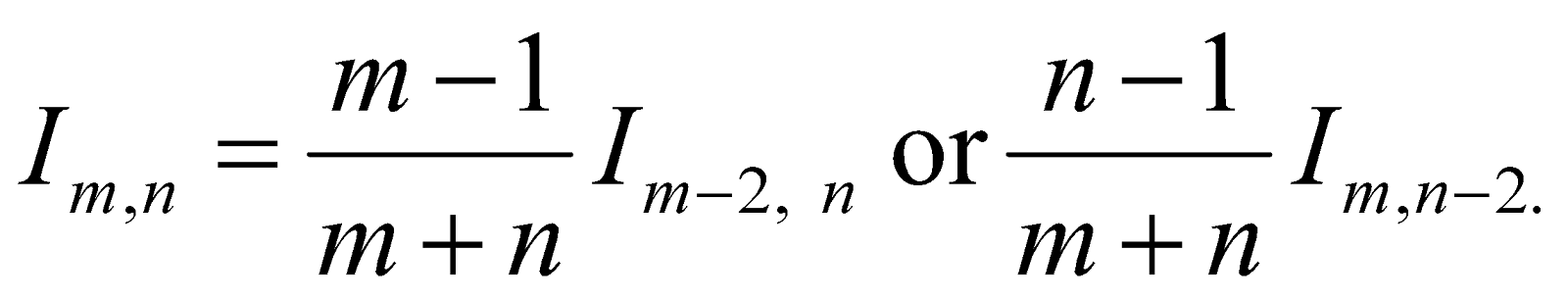Or
Find the length of the arc of the parabola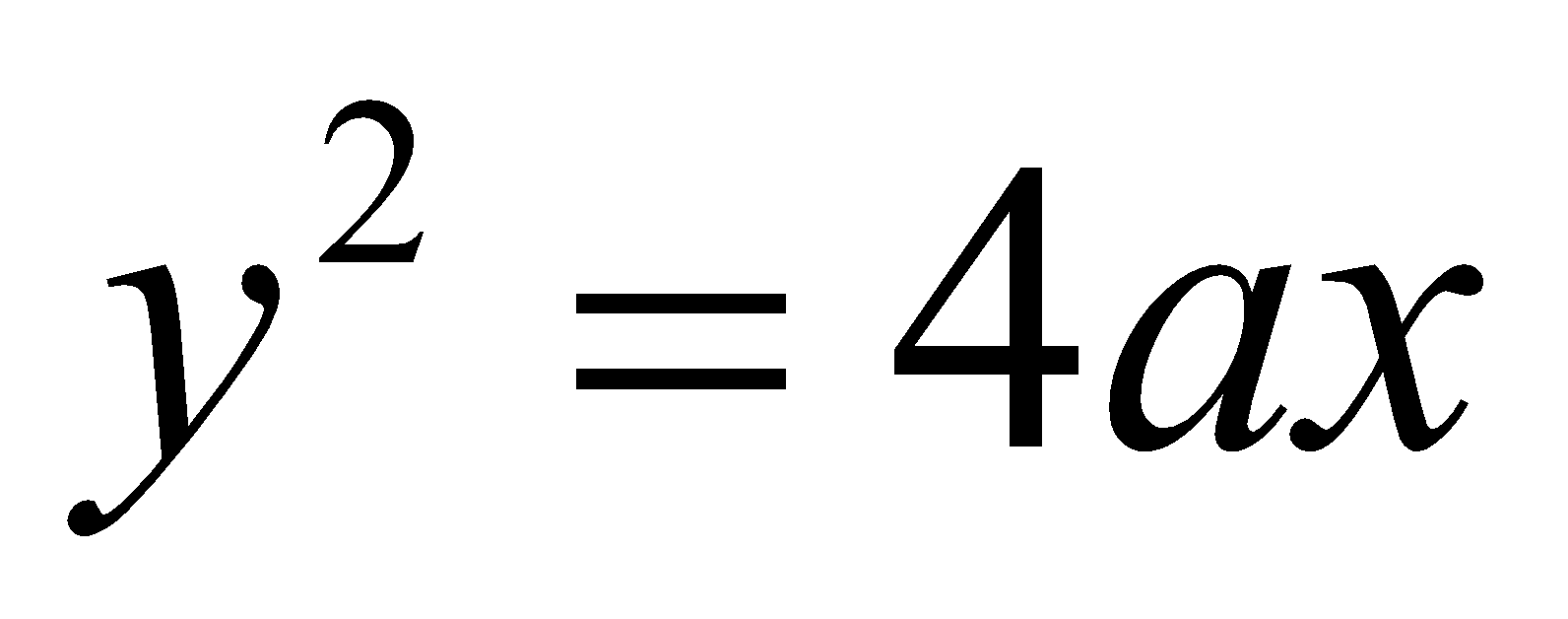cut-off by its latus rectum.
(c) Establish the reduction formula 2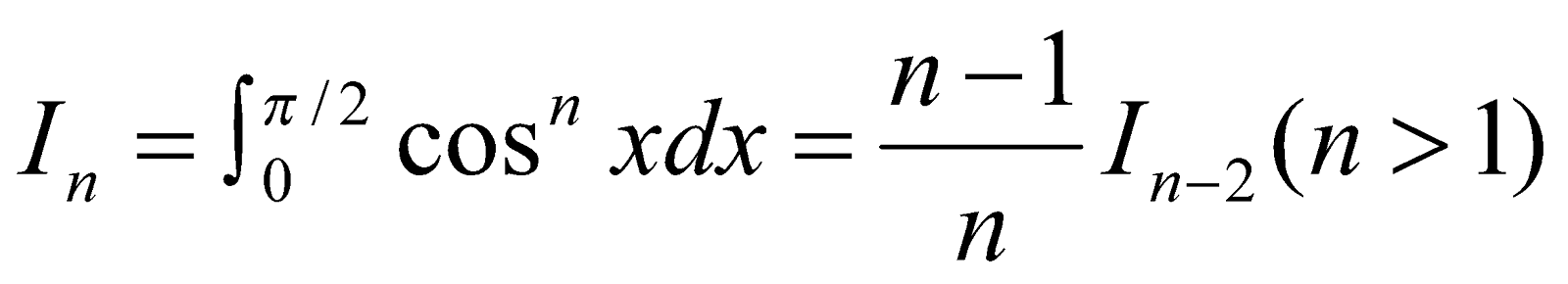where n is an integer.

***